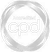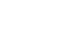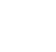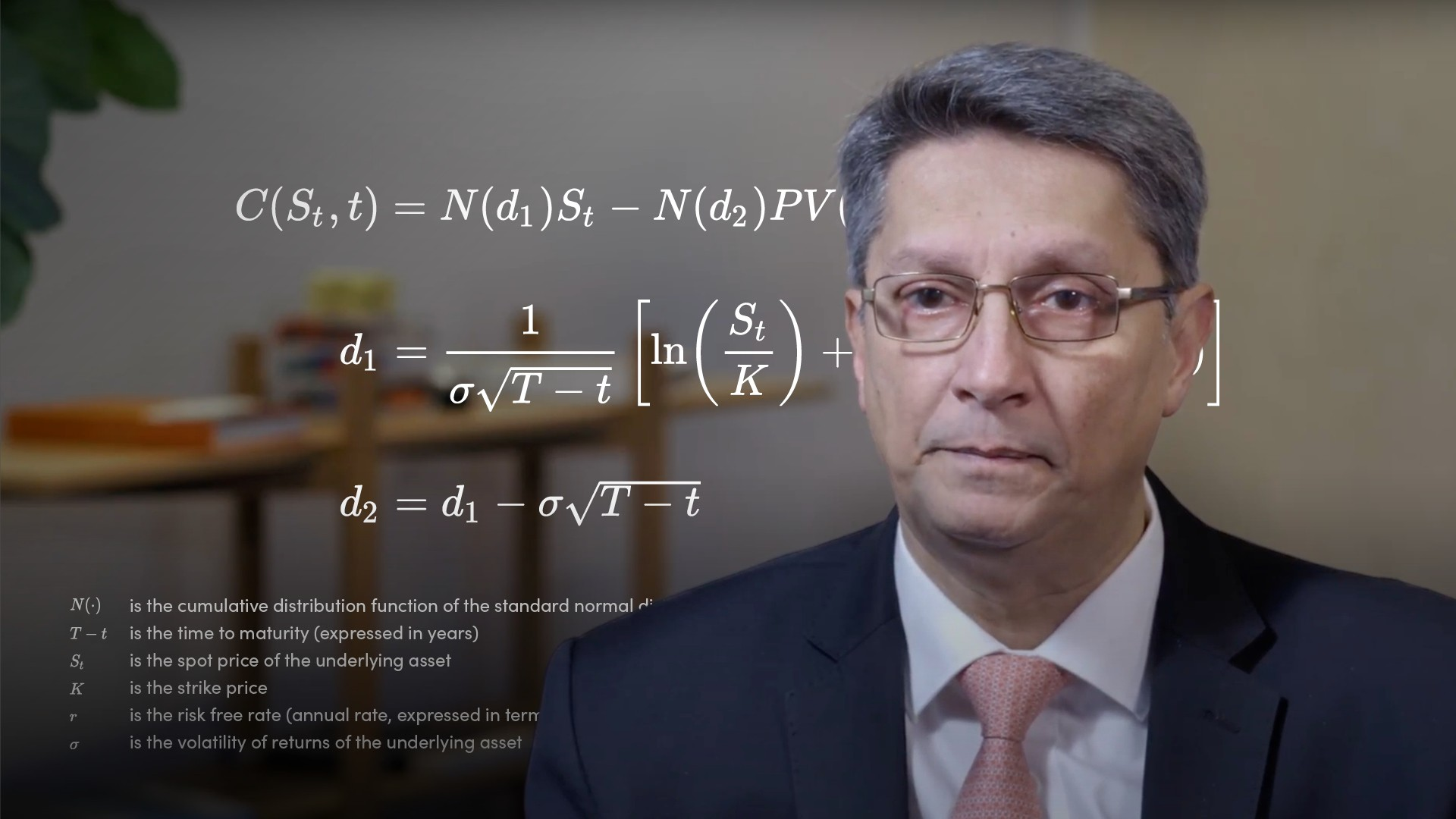# Option Pricing without Black-Scholes### Abdulla Javeri

The Black-Scholes Merton option pricing model is the Nobel Prize winning formula that is used as the basis of pricing options. In this video, Abdulla will discuss option pricing without Black-Scholes and in doing so it will provide hints to understanding the more complex area of option Greeks which will be covered in the second video on this topic.

Financial MathsThe Black-Scholes Merton option pricing model is the Nobel Prize winning formula that is used as the basis of pricing options. In this video, Abdulla will discuss option pricing without Black-Scholes and in doing so it will provide hints to understanding the more complex area of option Greeks which will be covered in the second video on this topic.

Finance Unlocked is the video learning platform built for finance professionals.### Option Pricing without Black-Scholes

5 mins 8 secs

Overview

Pricing an option requires a range of outcomes with the associated probability for each outcome, in other words, we need to make an assumption regarding the future probability distribution. We can work out the total probability weighted payoff based on our assumptions, which when discounted gives us the option price.

Key learning objectives:

• Outline the Black-Scholes formula

• Describe an option payoff

• Understand how we price an option

## Book a demo to access

Summary

#### What does the Black-Scholes formula allow us to do?

The Black-Scholes Merton option pricing model is probably the most celebrated formula in the world of options and is still in use as the basis of pricing options. The formula allows us to price options simply by inputting numbers in the relevant spaces

#### What is the Black-Scholes formula?#### What is an option payoff?

We would only exercise an option if it had a positive value at expiry, a positive payoff, irrespective of the premium that we originally paid for it.

For a call option, the payoff would be the maximum of the spot at expiry minus the strike, or zero. For a put option, it would be the maximum of the strike minus the spot at expiry, or zero. For example, if we take a strike of 500 and the expiry price of 520, the call would be exercised as it would have a payoff of 20. Whereas the put would be abandoned as the payoff would be zero, the maximum of -20 or zero.

#### How can we calculate the total probability weighted payoff/price of an option in the following example?

• Assume the option has a strike of 100, with one year to expiry. Let’s say that we could look into the future and know for certain that the price at expiry will be 110, the option payoff would be the maximum of the spot minus the strike (110-100 or zero) = a payoff of 10.
• The probability weighted playoff is the payoff multiplied by the probability. The payoff will be received in a year, so the call price today will be the present value of the expected payoff. Hence, we’ve used continuous rates and discounting.
• Let’s also assume that there are two possible price outcomes; 80 and 110, and arbitrarily assign a 50% probability to each outcome. Half the time the payoff is zero, and half the time the payoff is 10. Multiply each payoff by 50% and add, giving a probability weighted payoff of 5, and then discount for the call price today, which gives us the option price
• Call/Put Price = 5 x e-(0.1 x 1) = 4.52

## Book a demo to access

Expert### Abdulla Javeri

Abdulla’s career in the financial markets started in 1990 when he entered the trading floor of the London International Financial Futures Exchange, LIFFE, and qualified as a pit trader in equity and equity index options. In 1996, Abdulla became a trainer for regulatory qualifications and then for non-exam courses, primarily covering all major financial products.03:18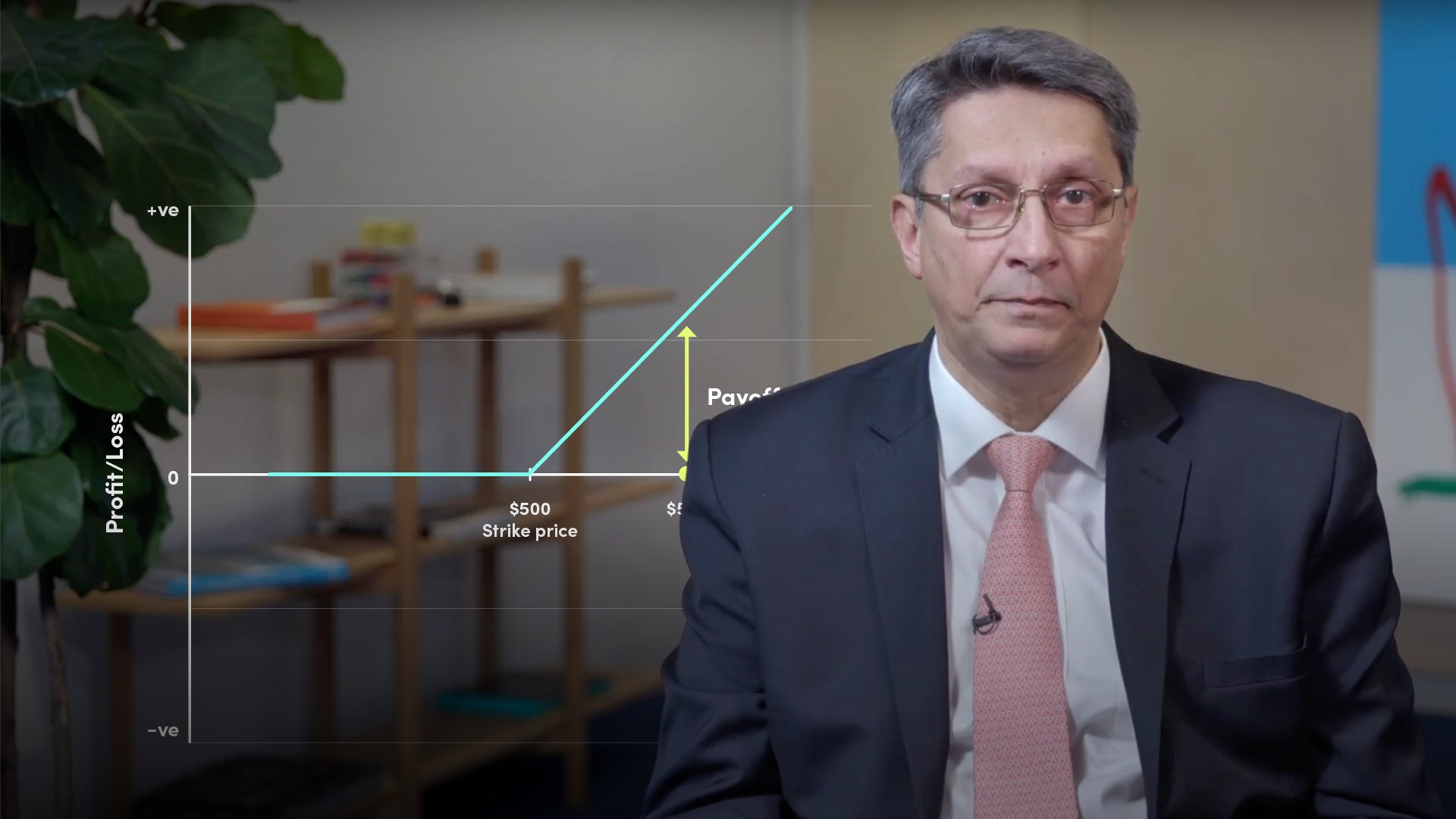05:12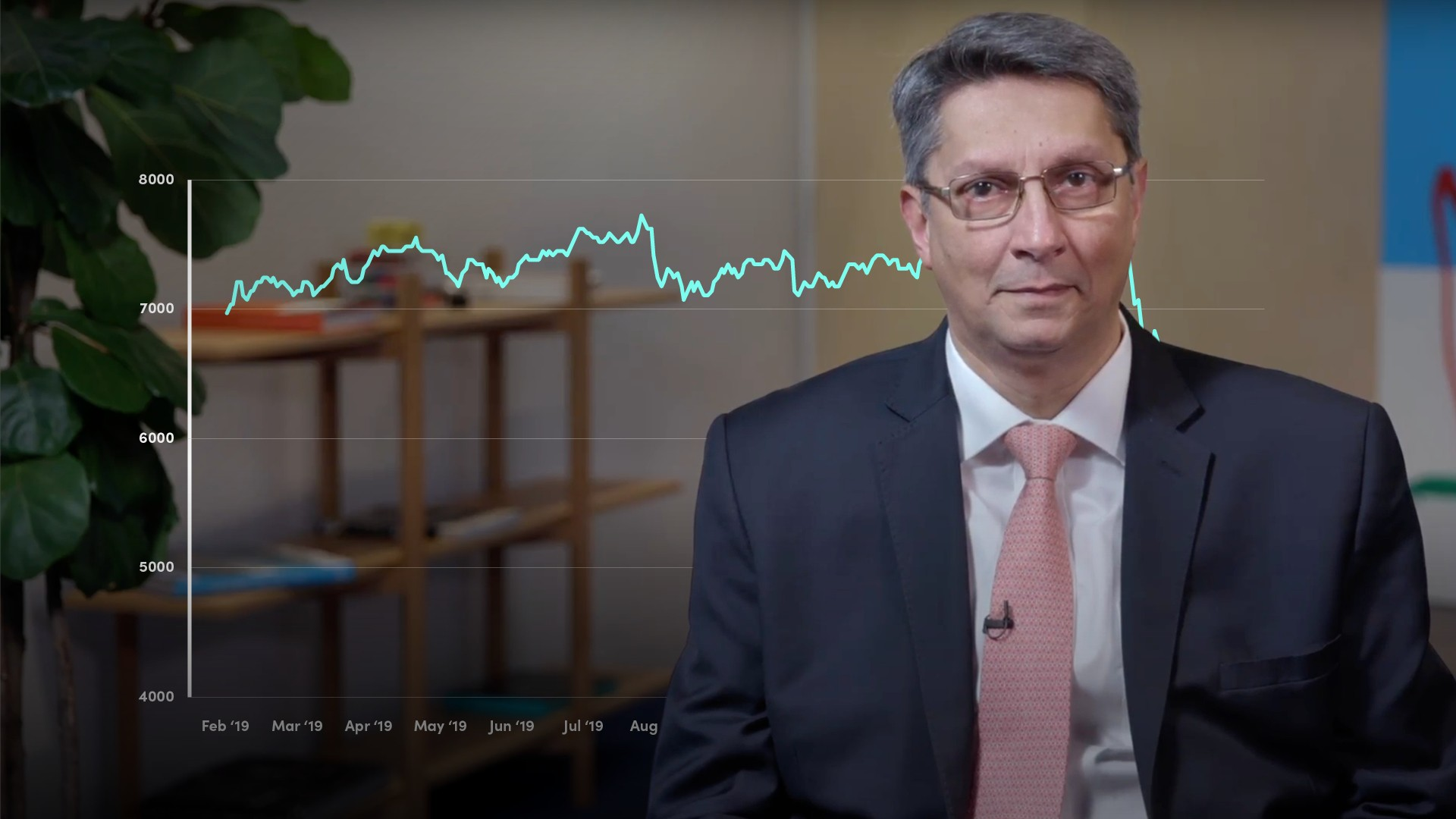05:39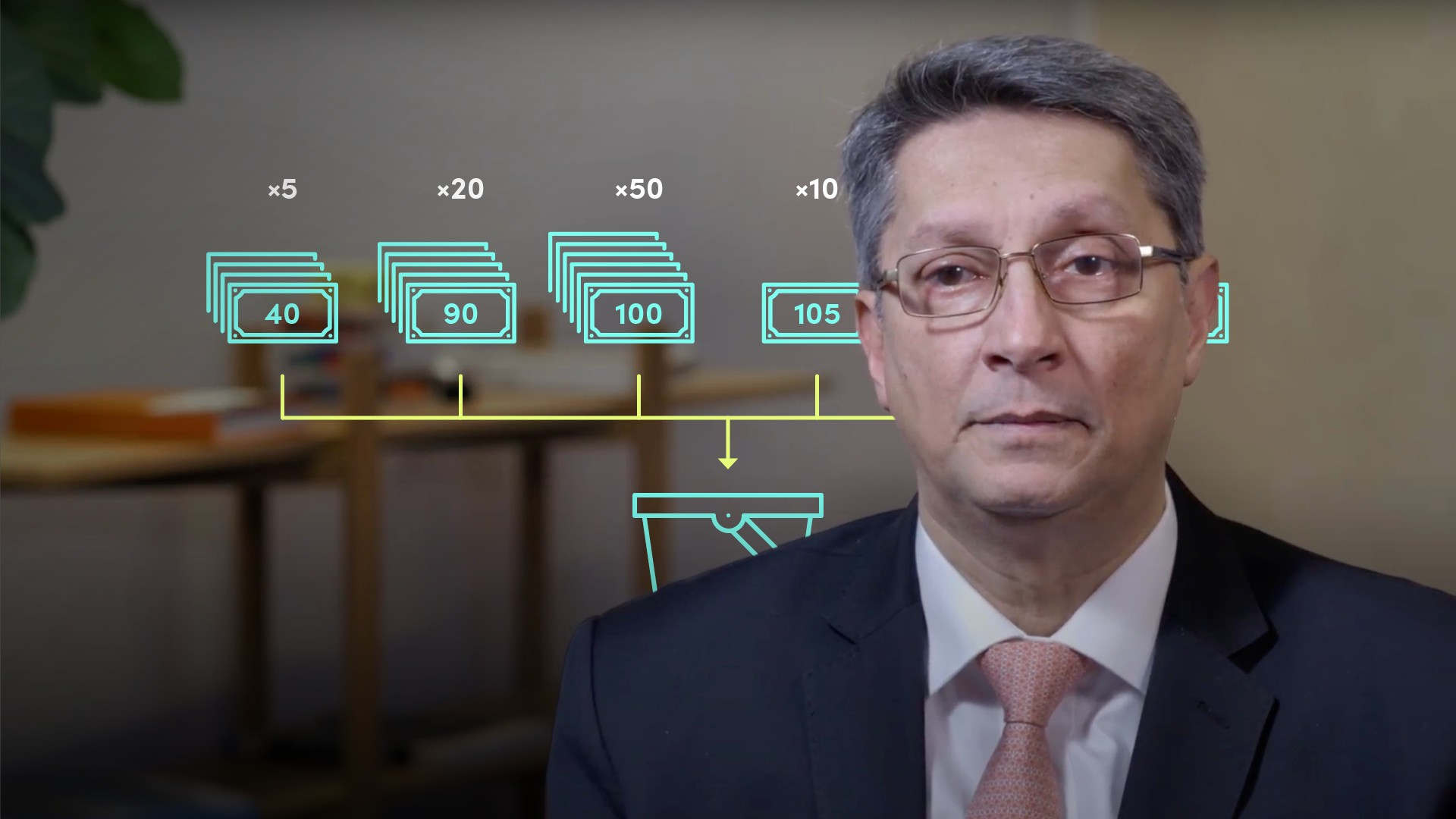04:52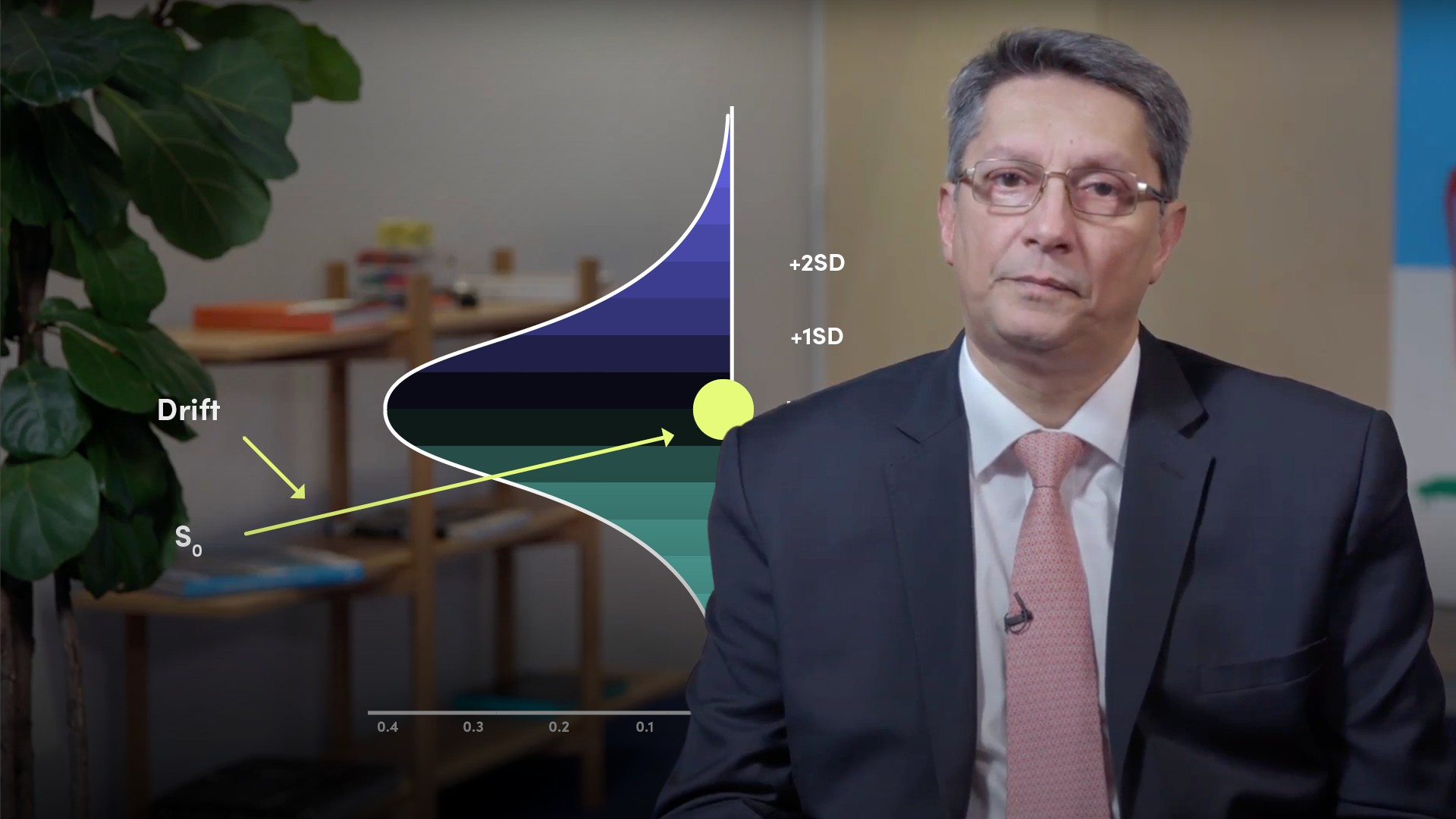05:09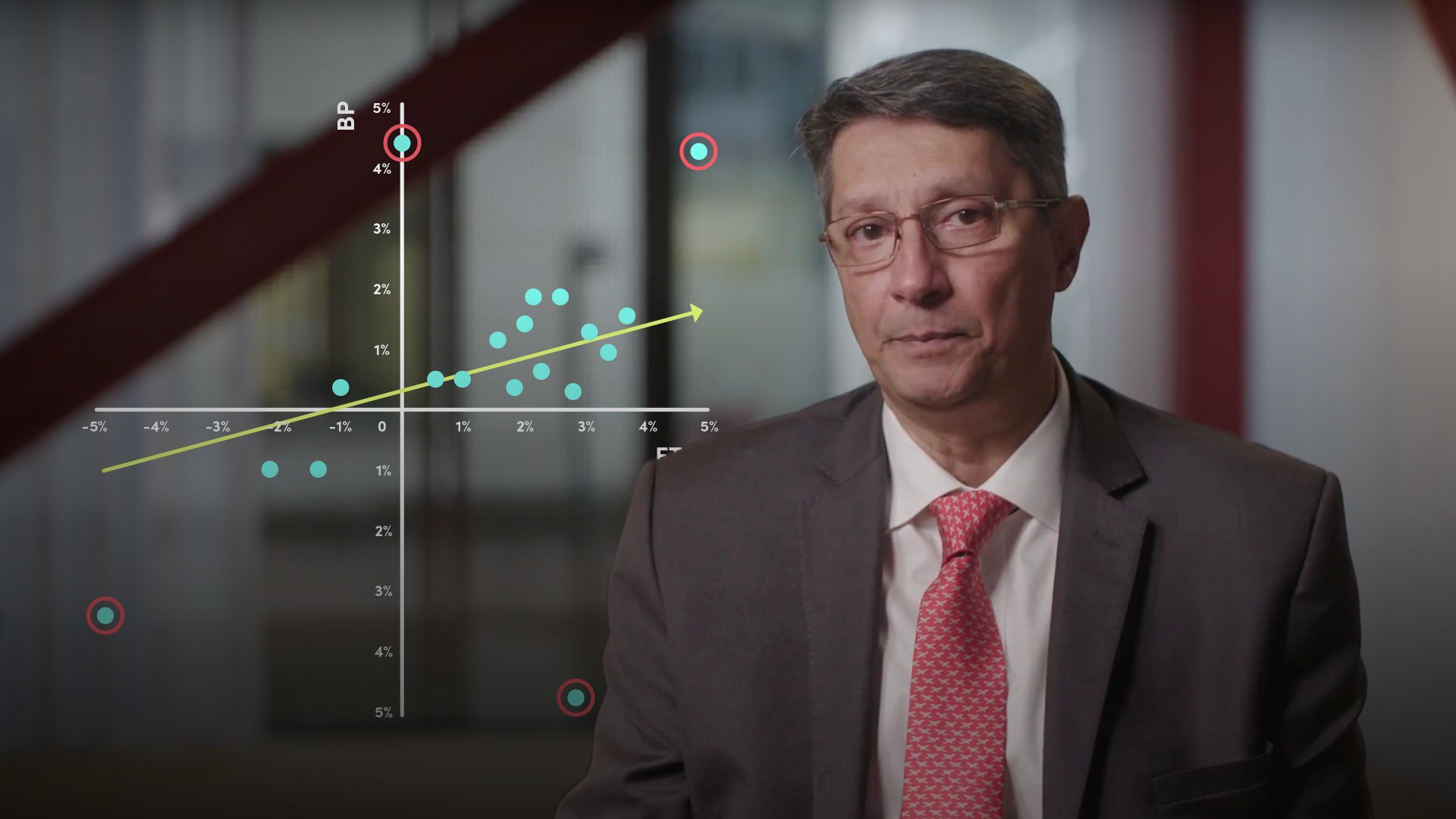04:1305:3804:08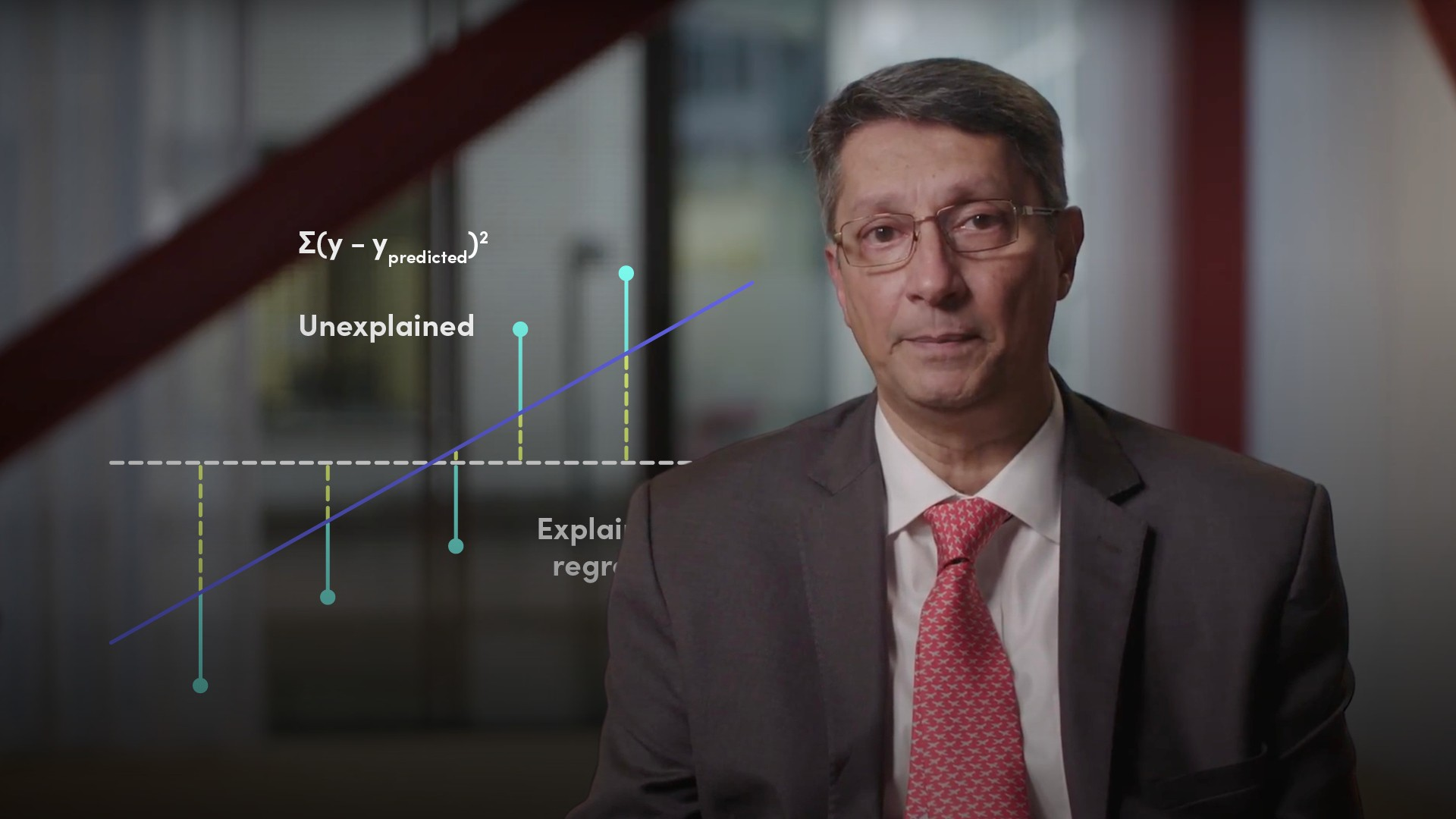04:2205:02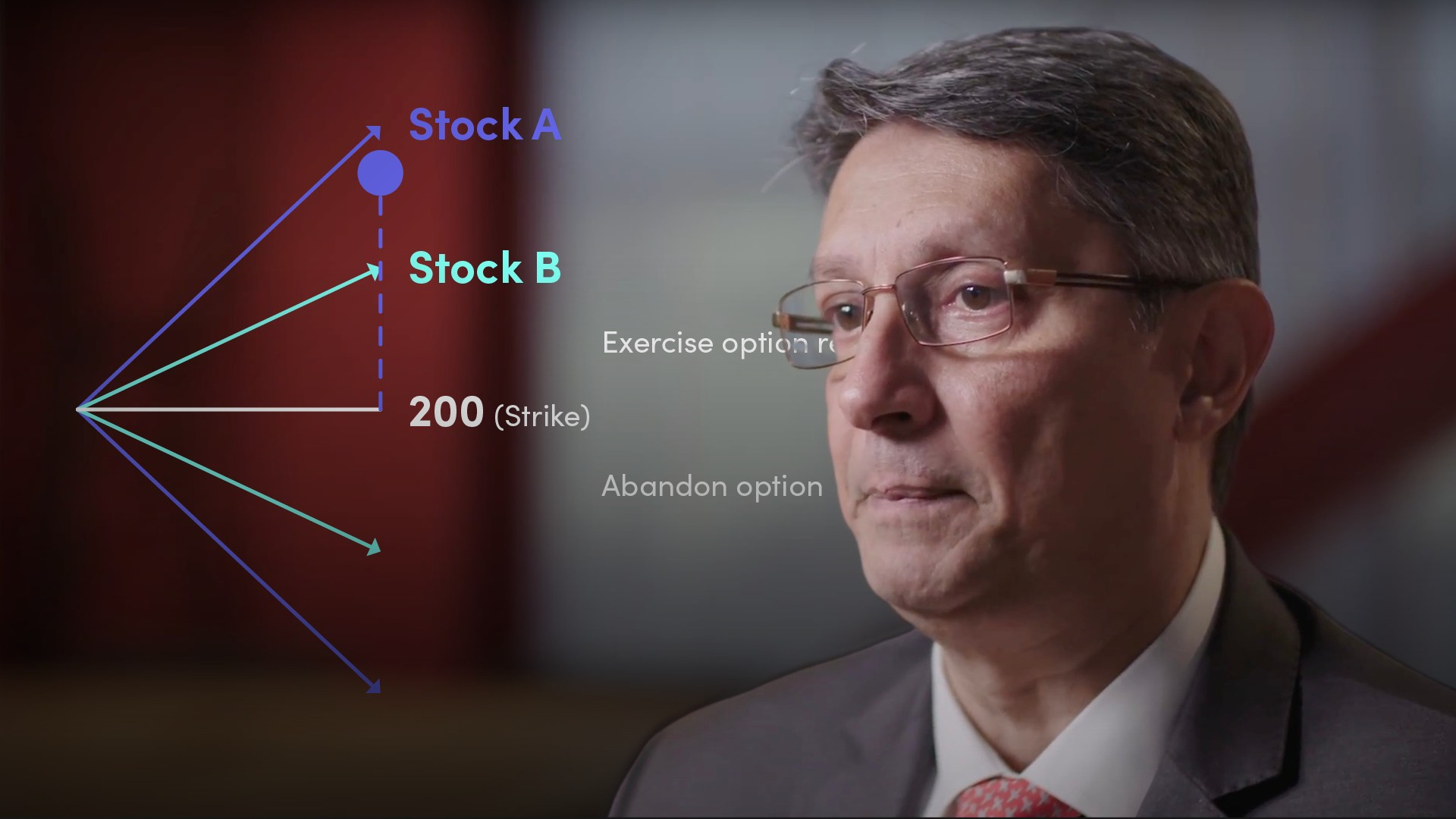03:26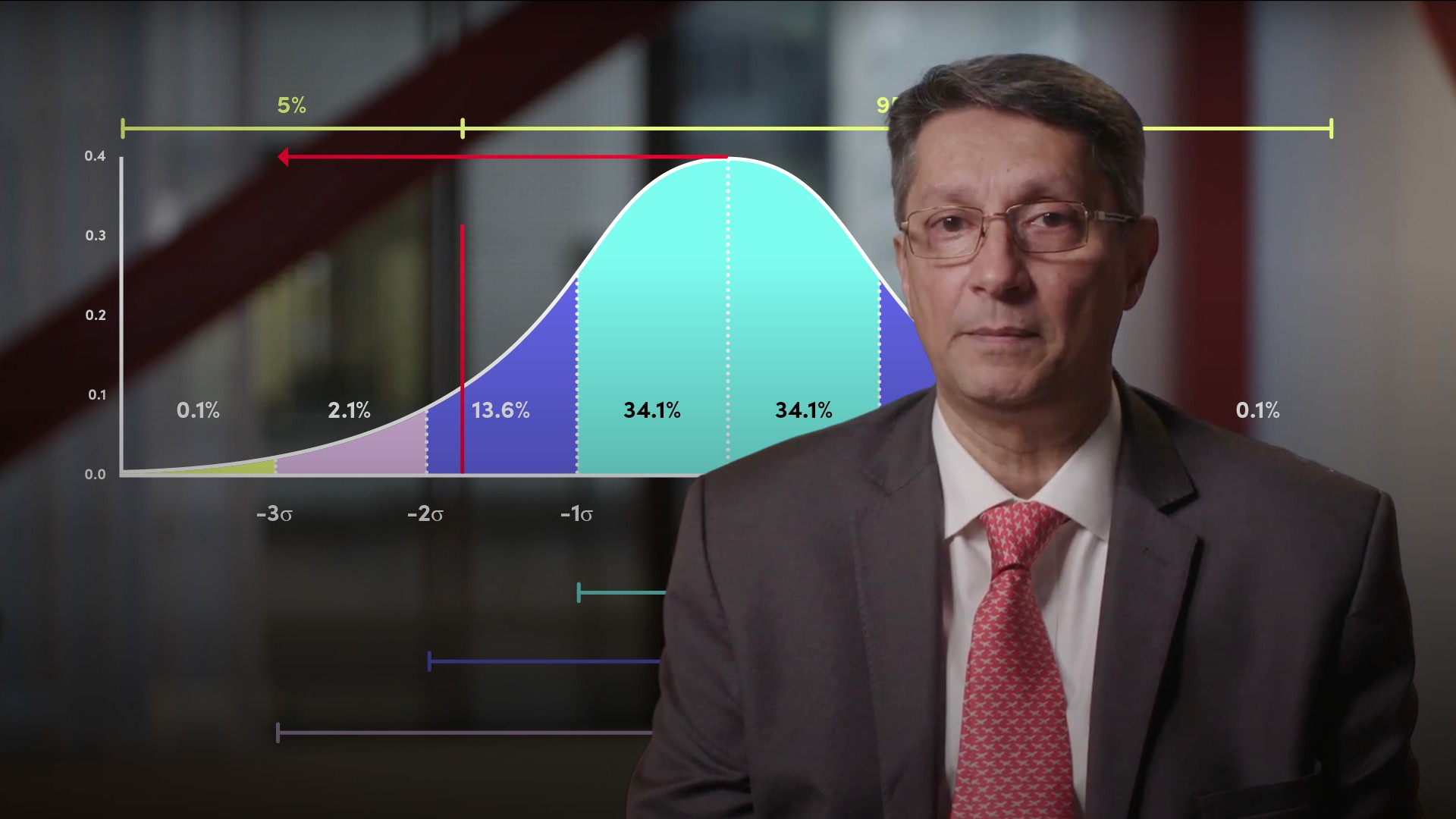03:58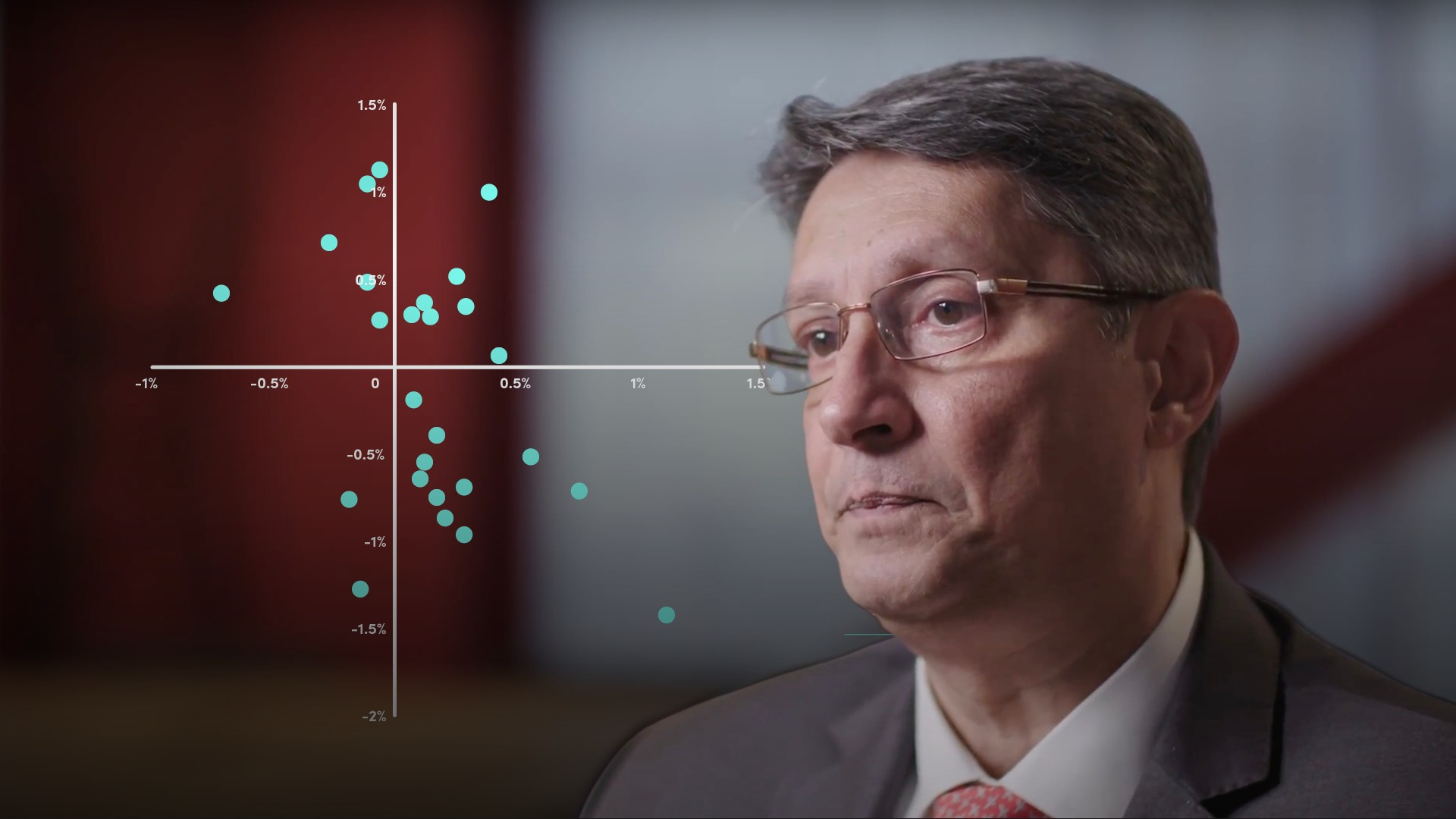04:55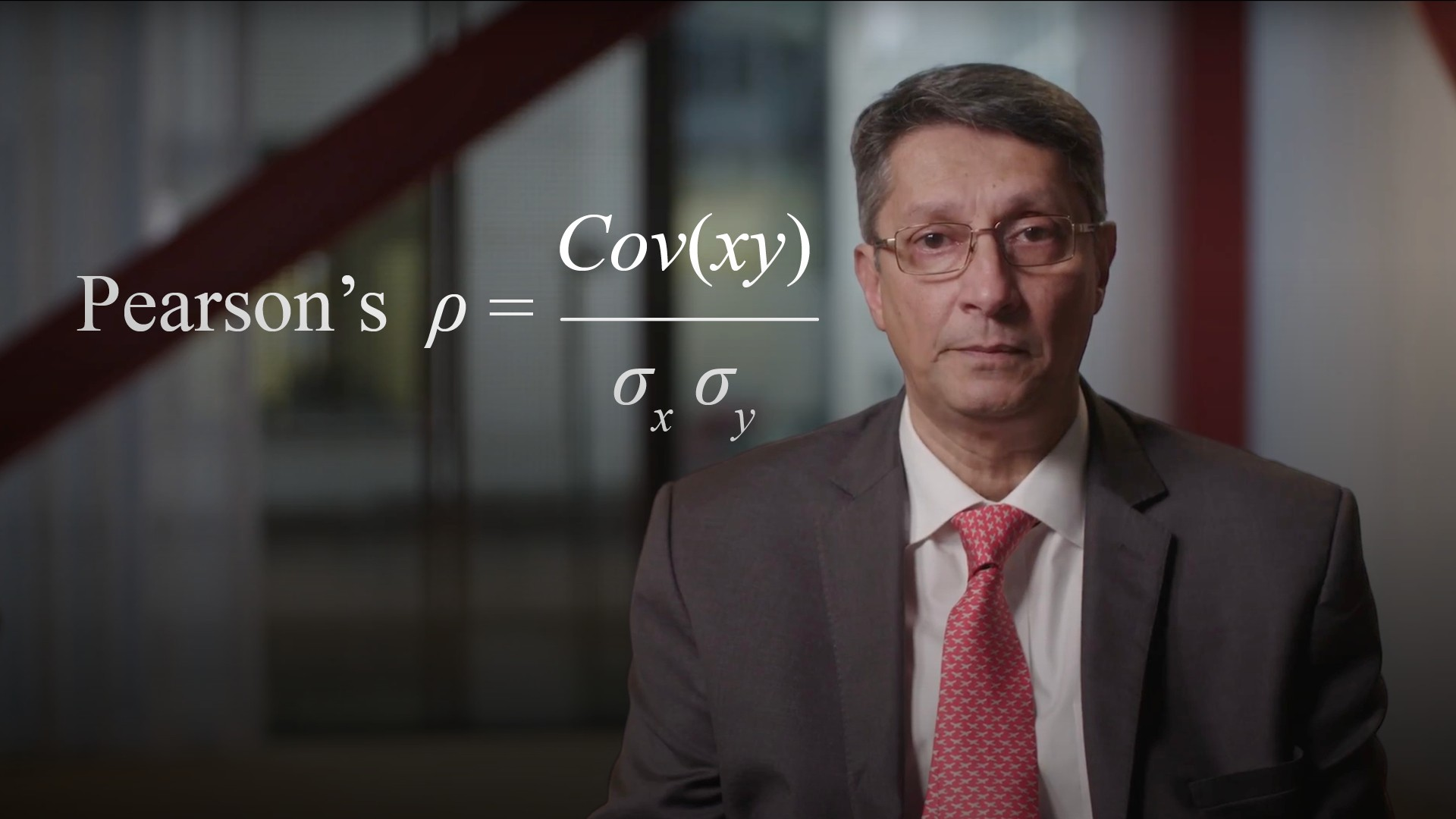04:56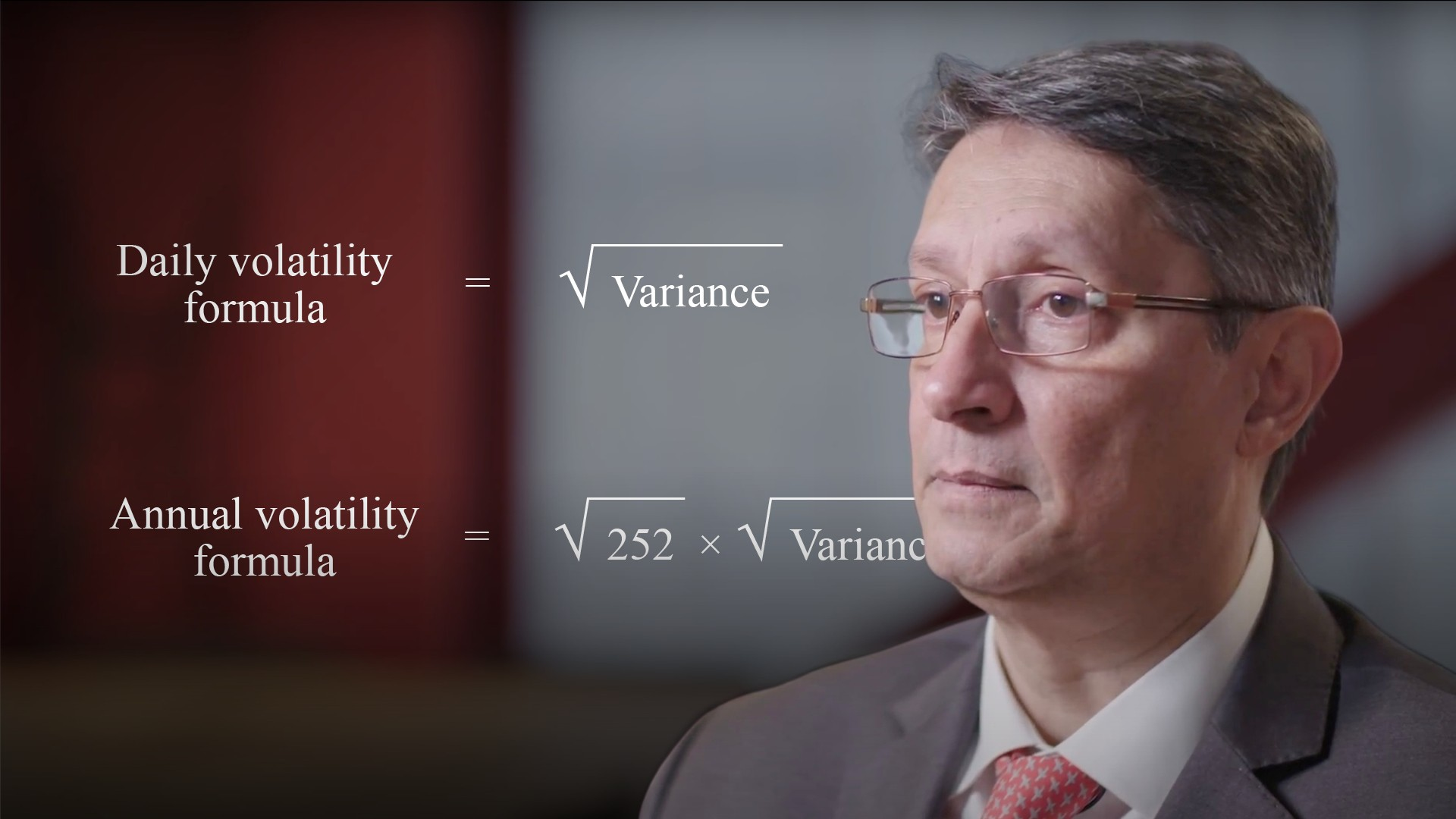04:34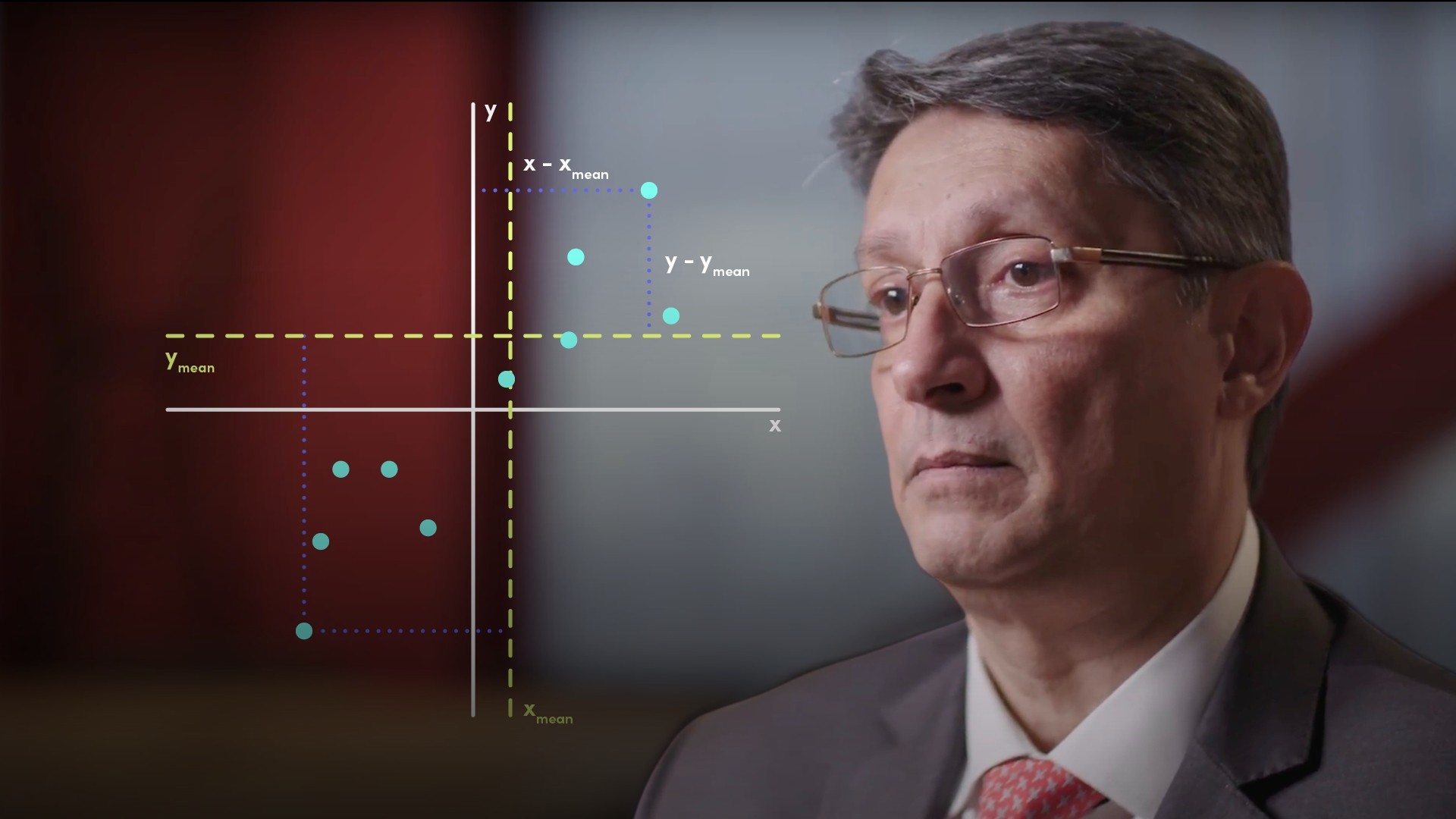04:40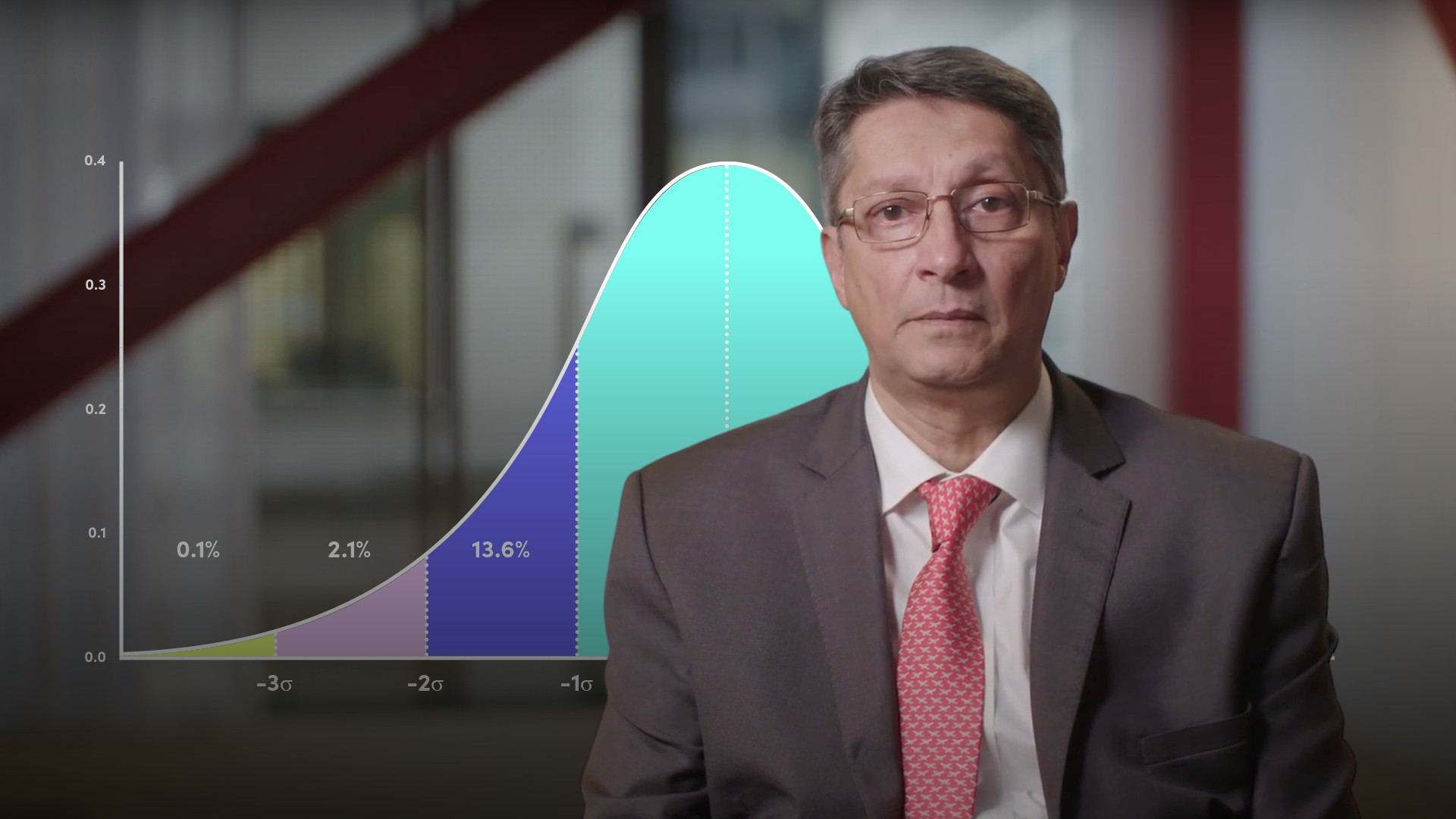05:42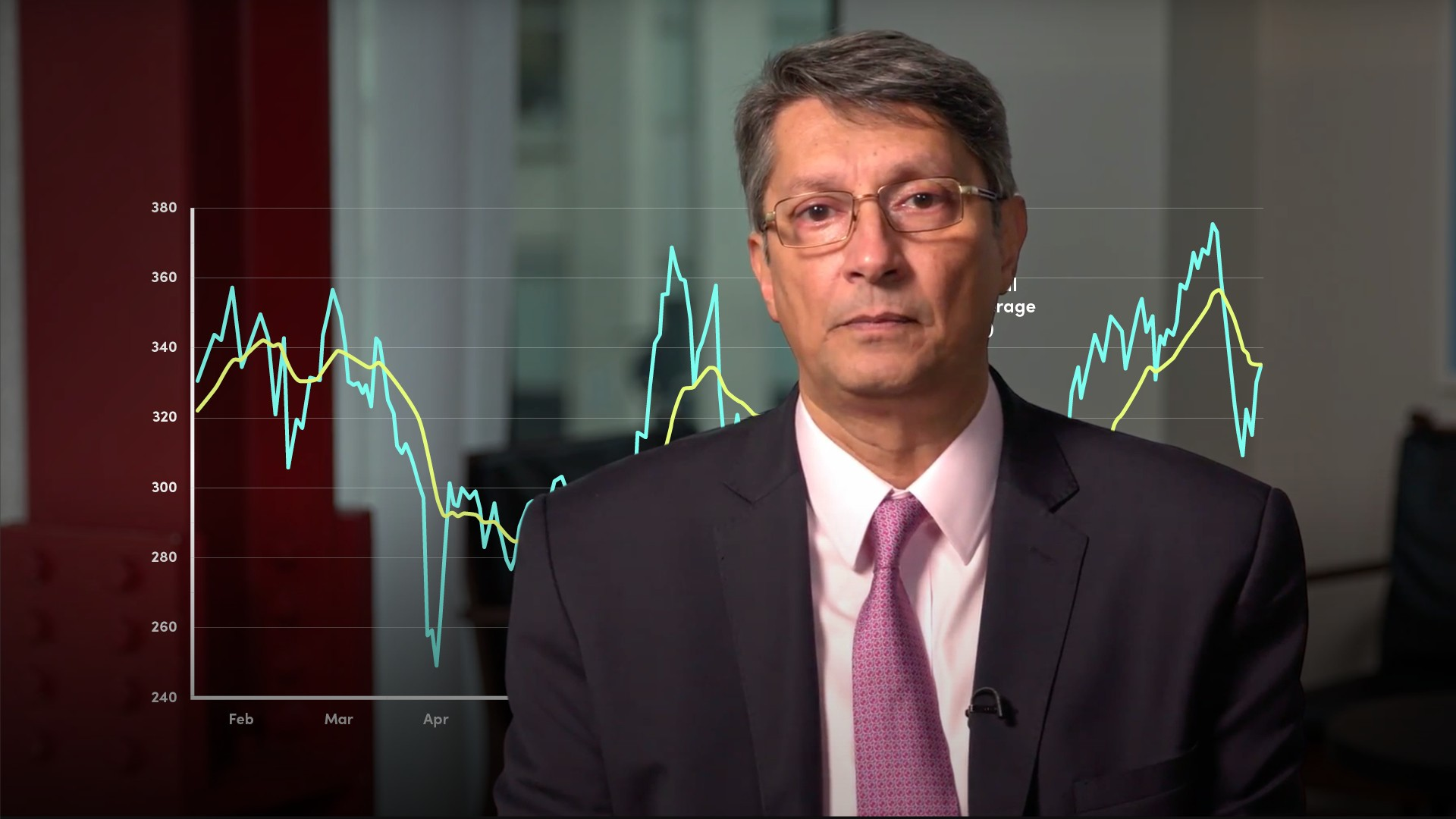05:11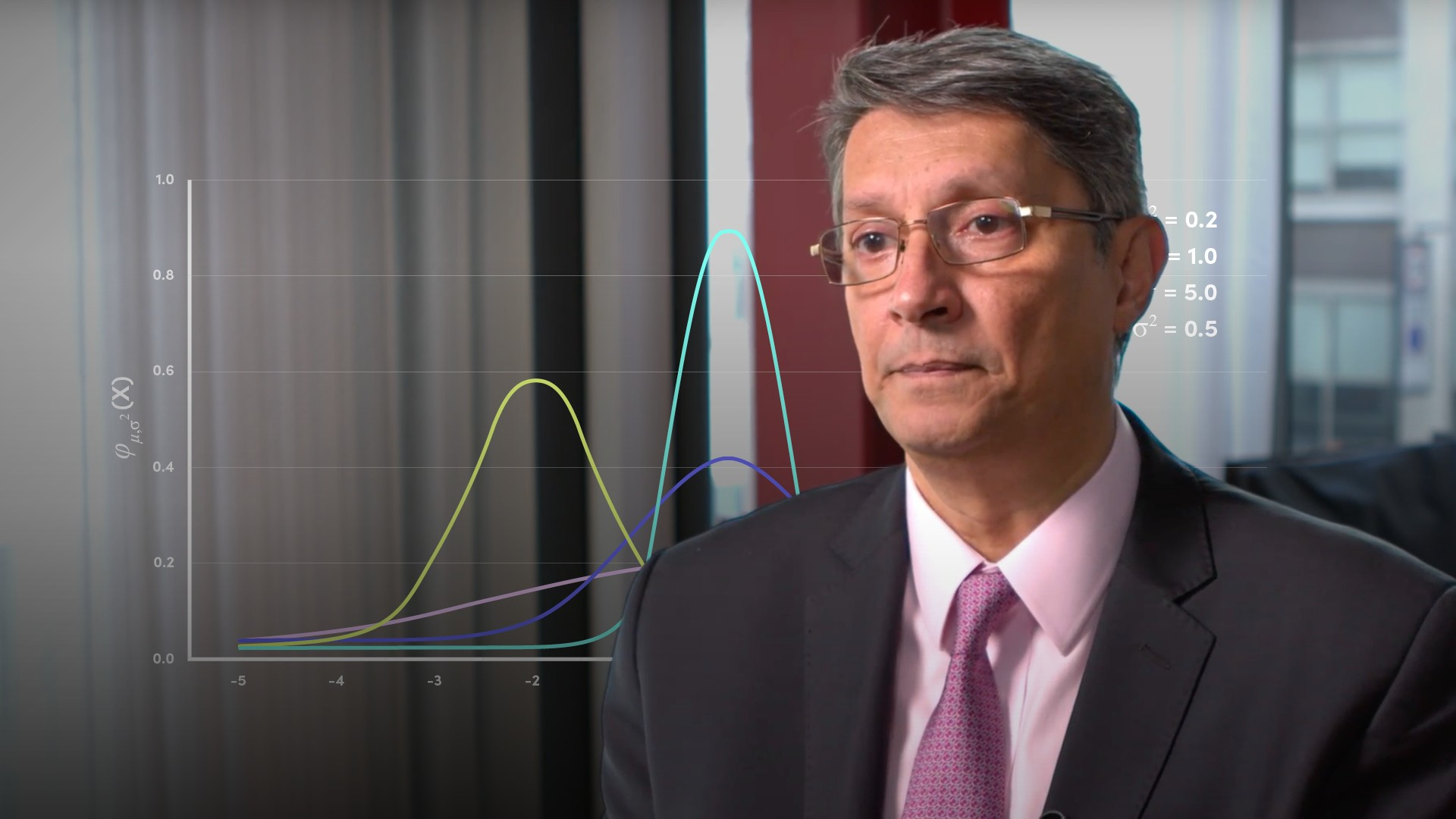04:49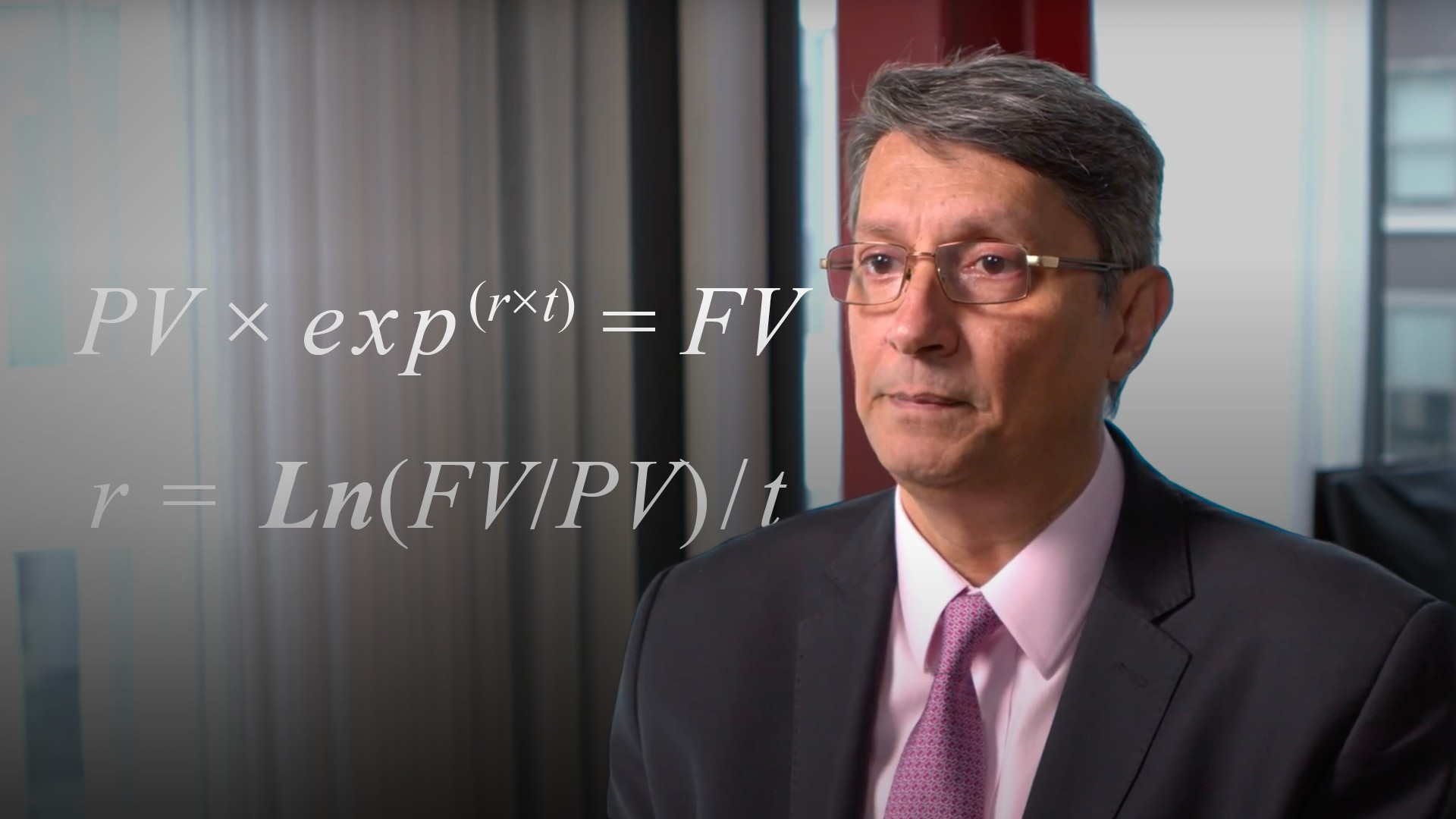03:5806:4403:49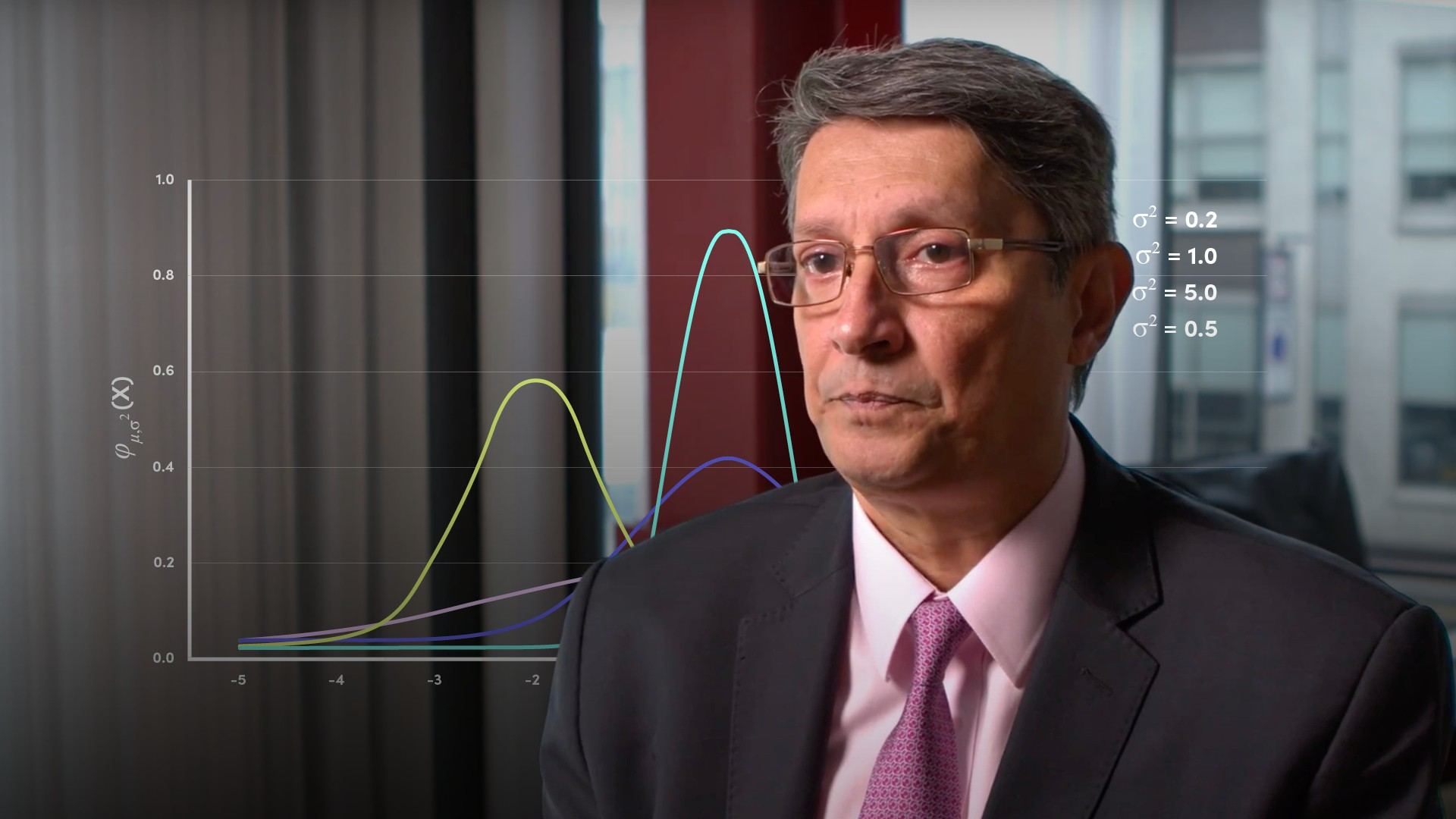04:57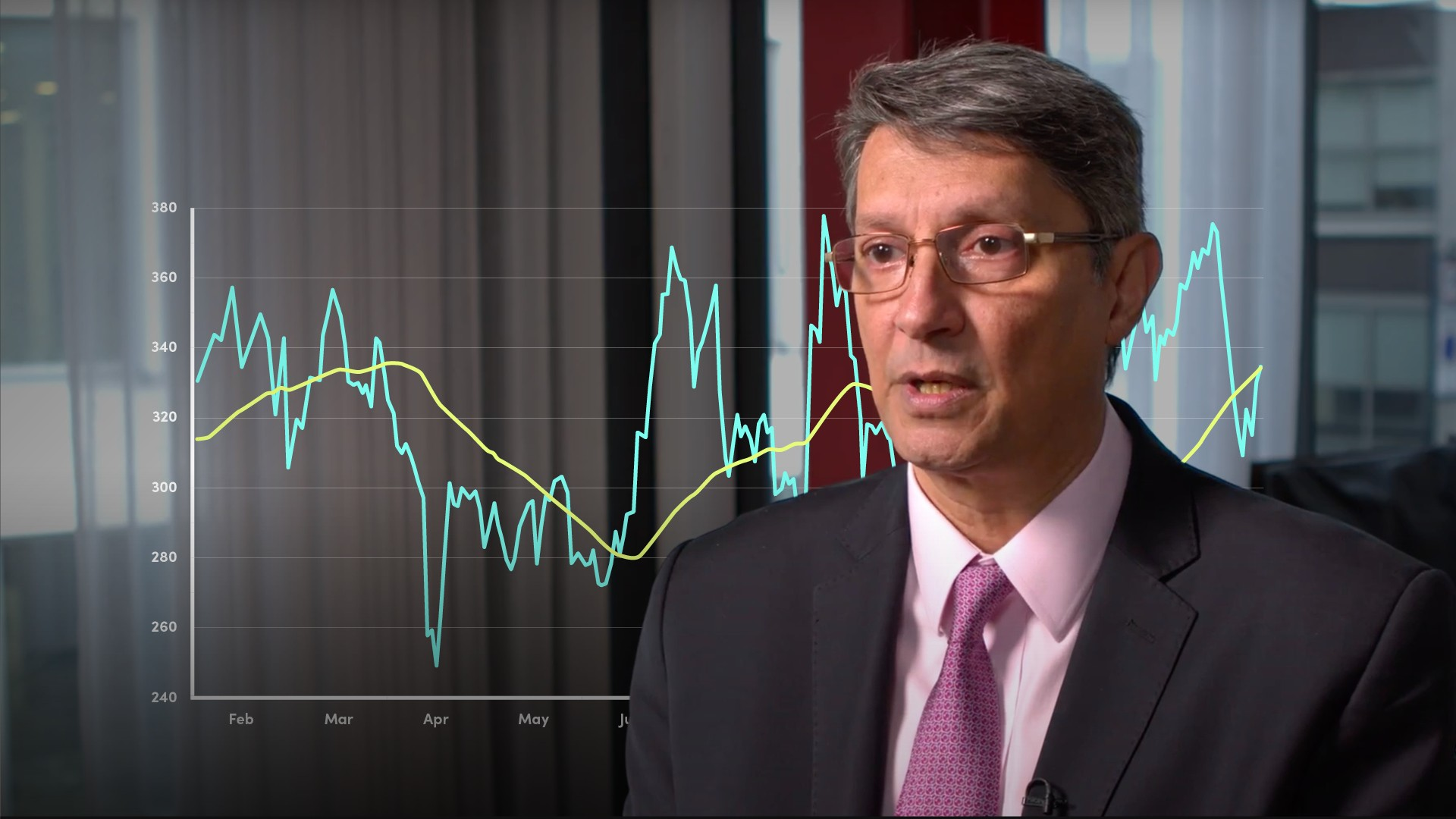04:0004:11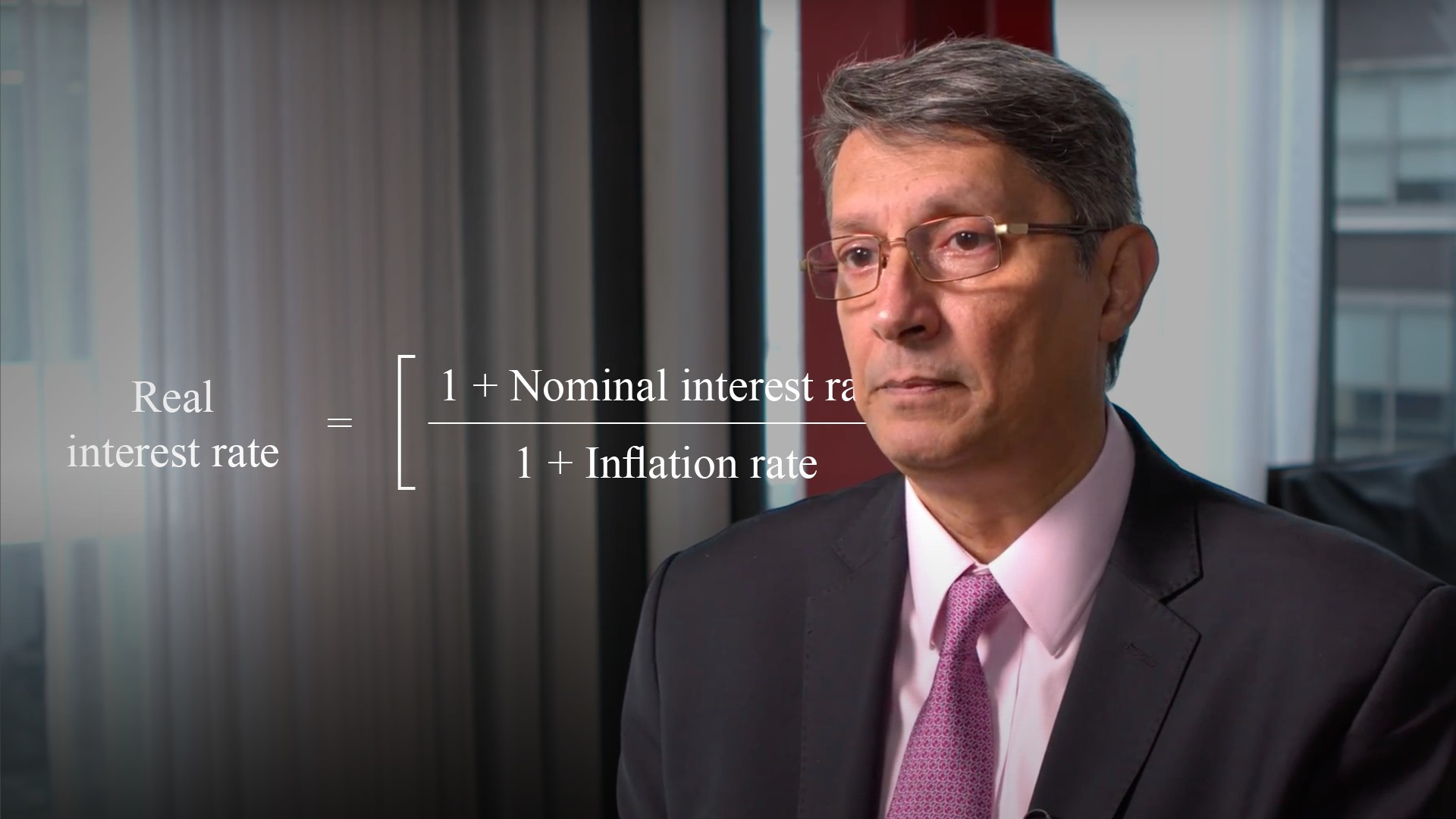04:42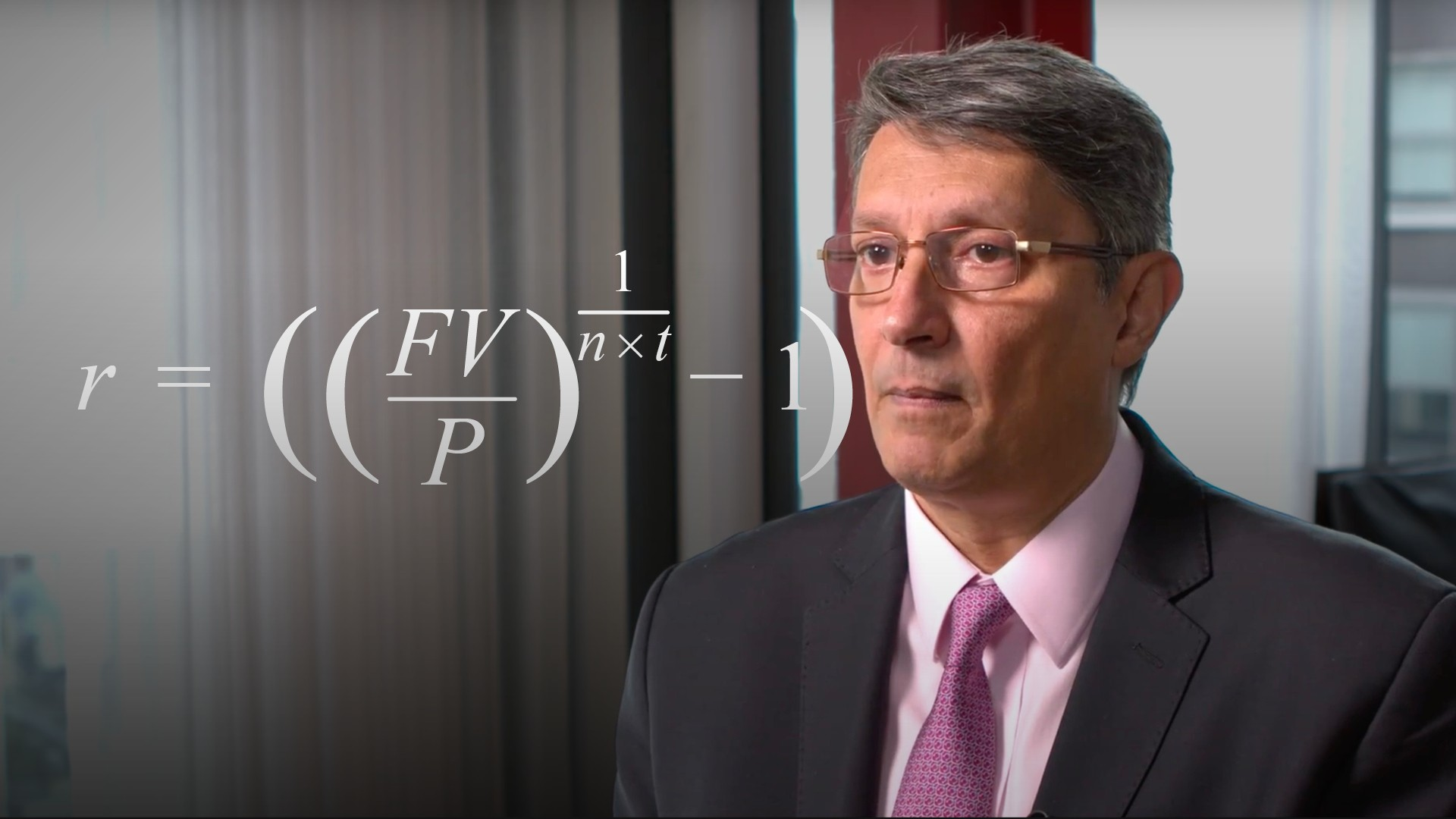05:03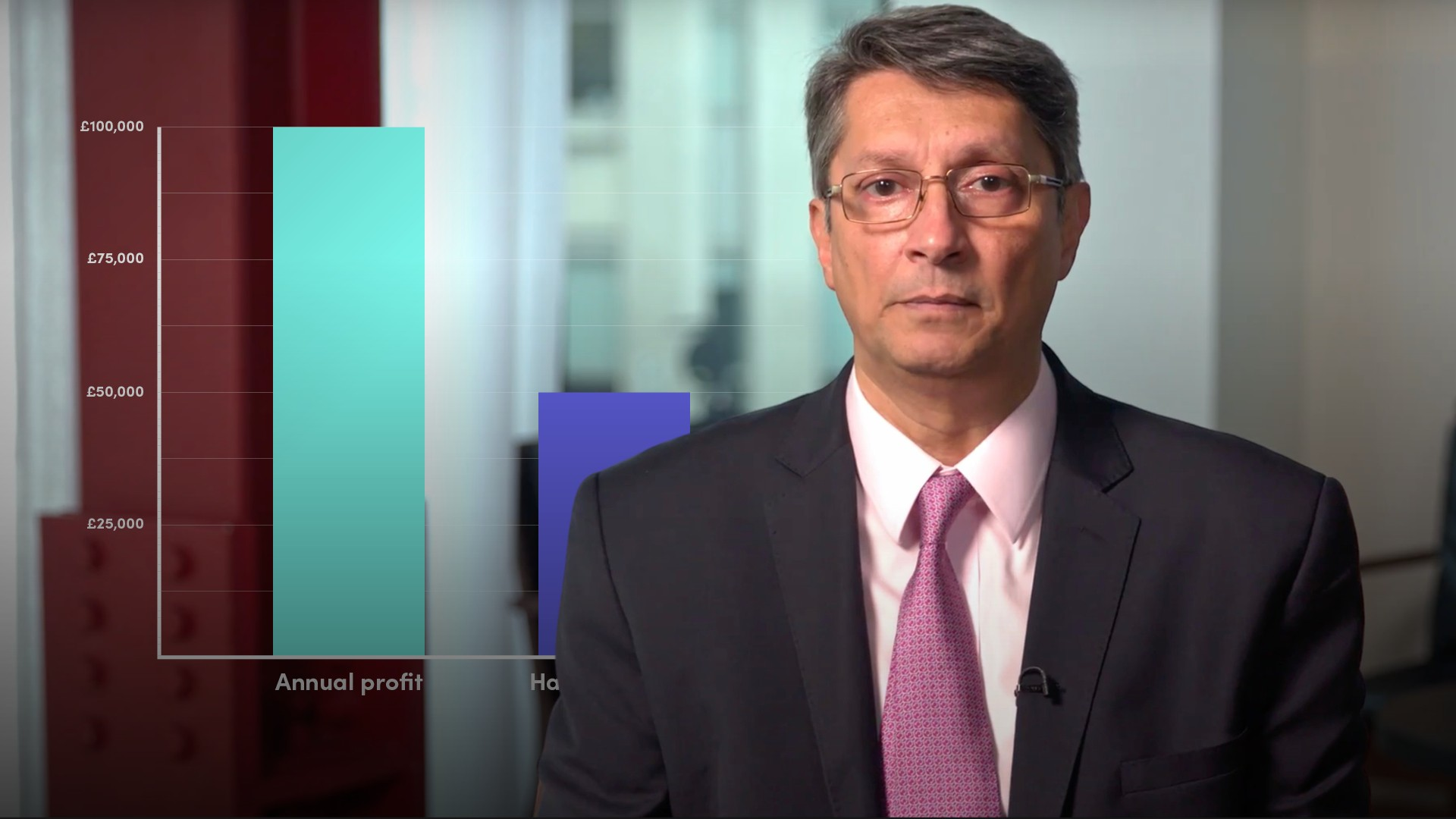04:37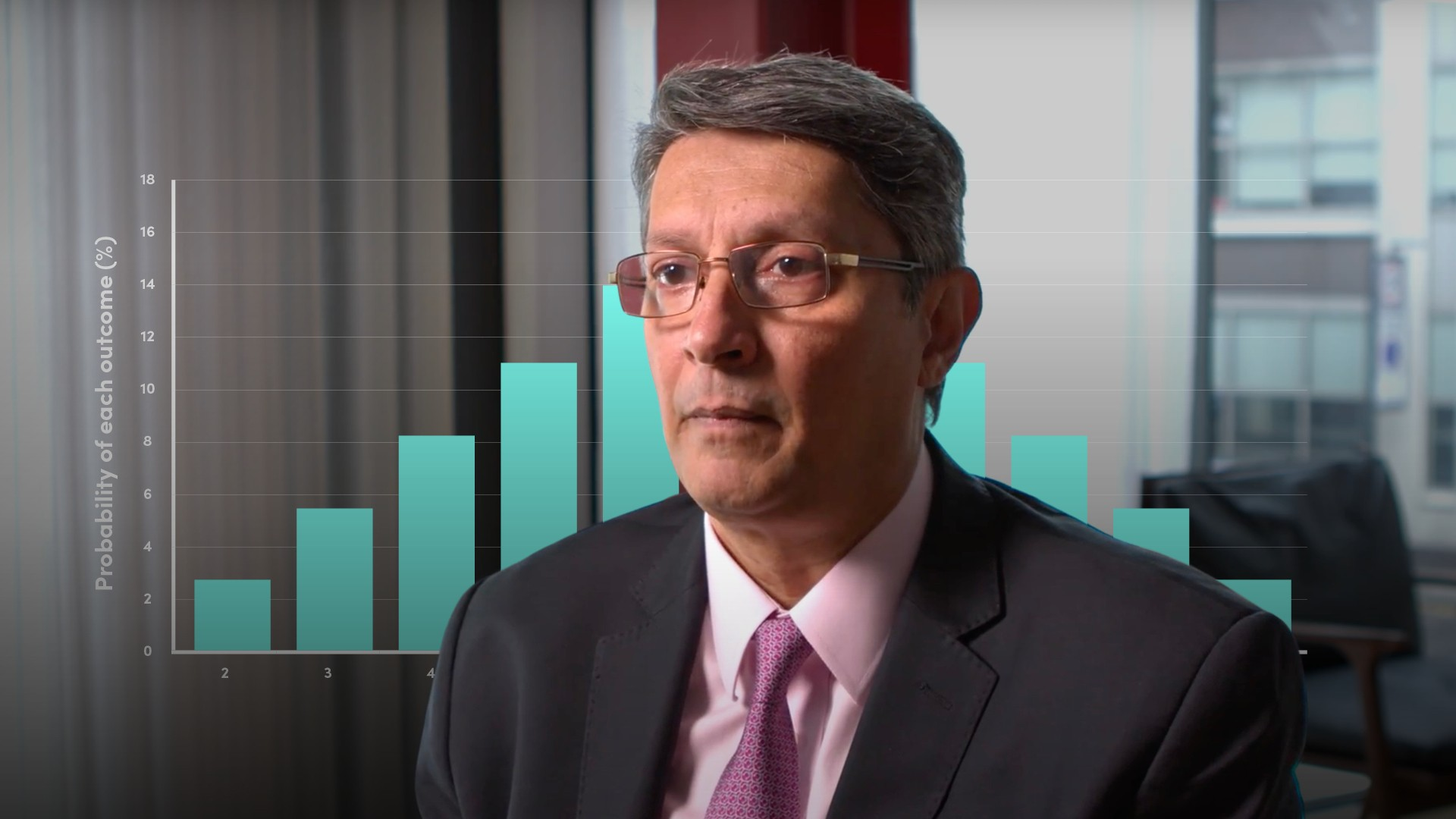04:36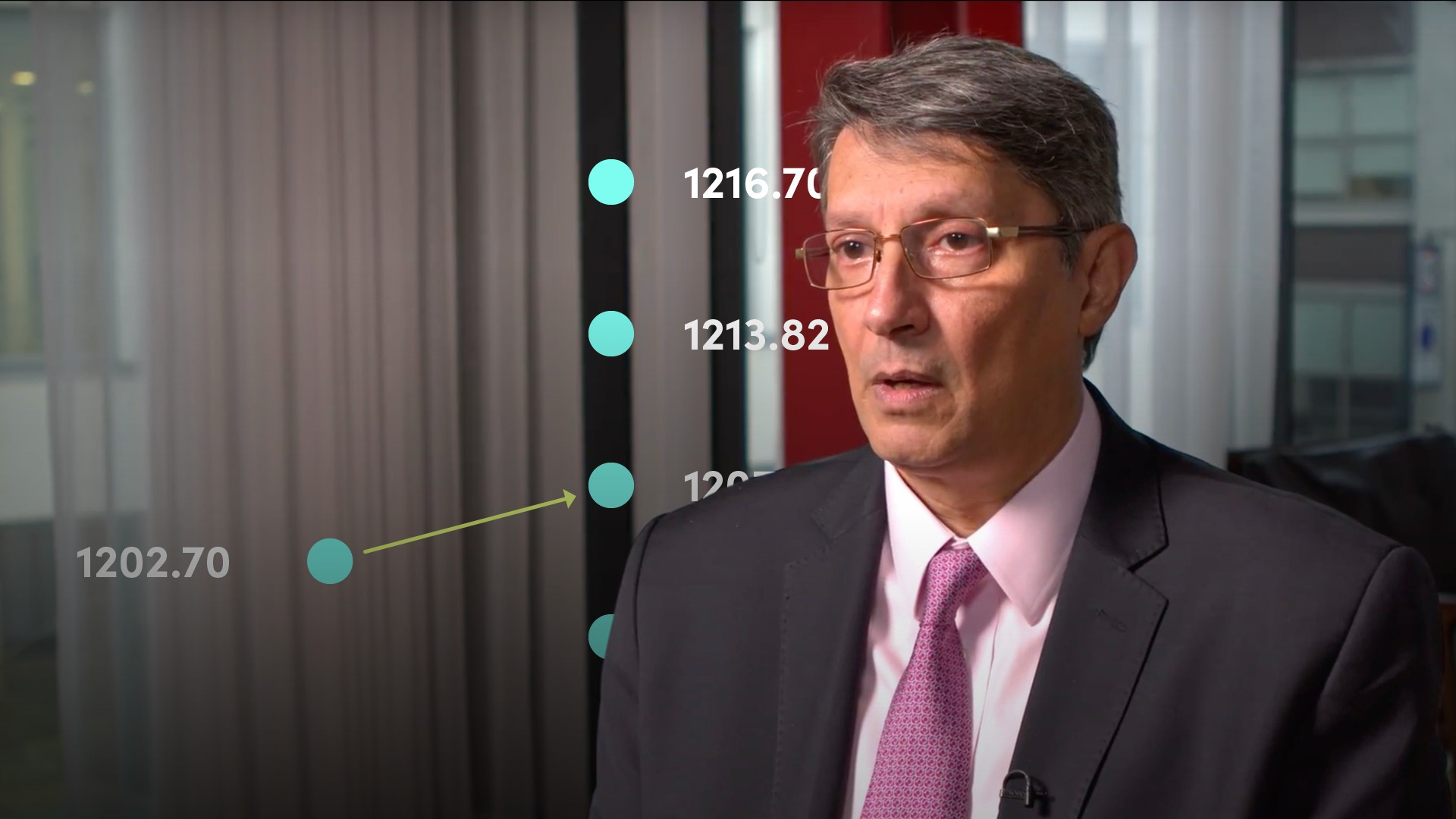06:35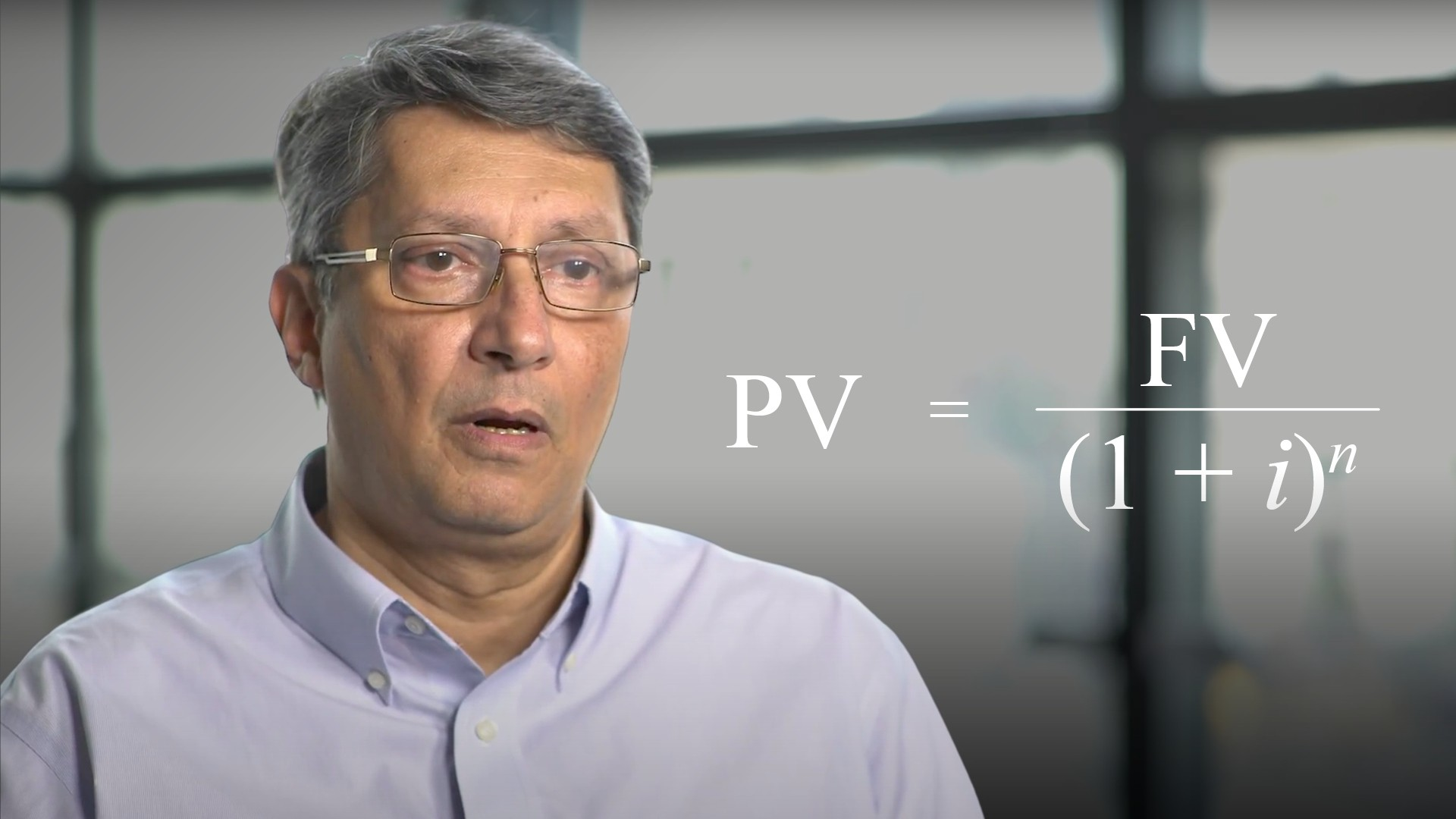05:00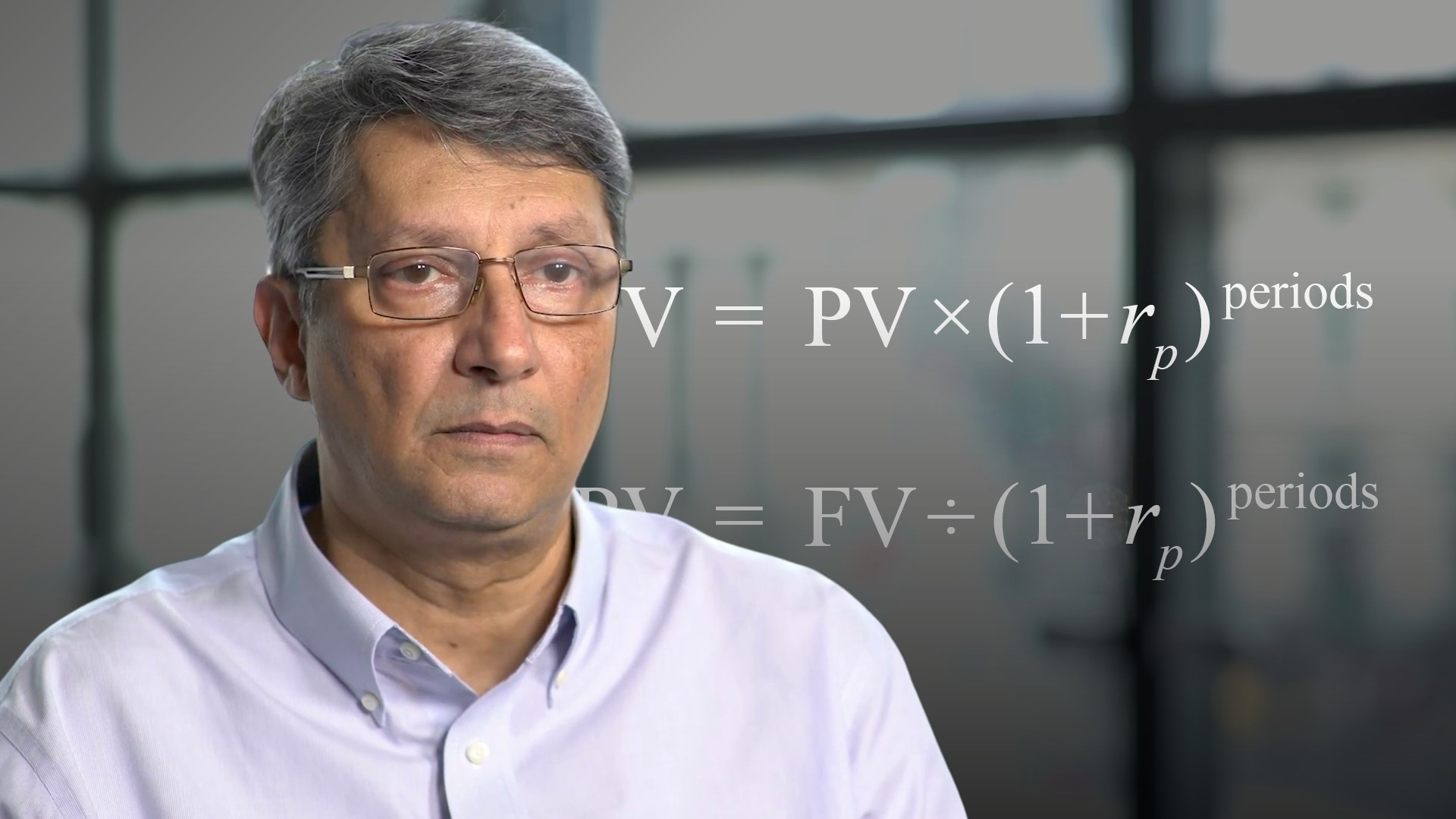03:10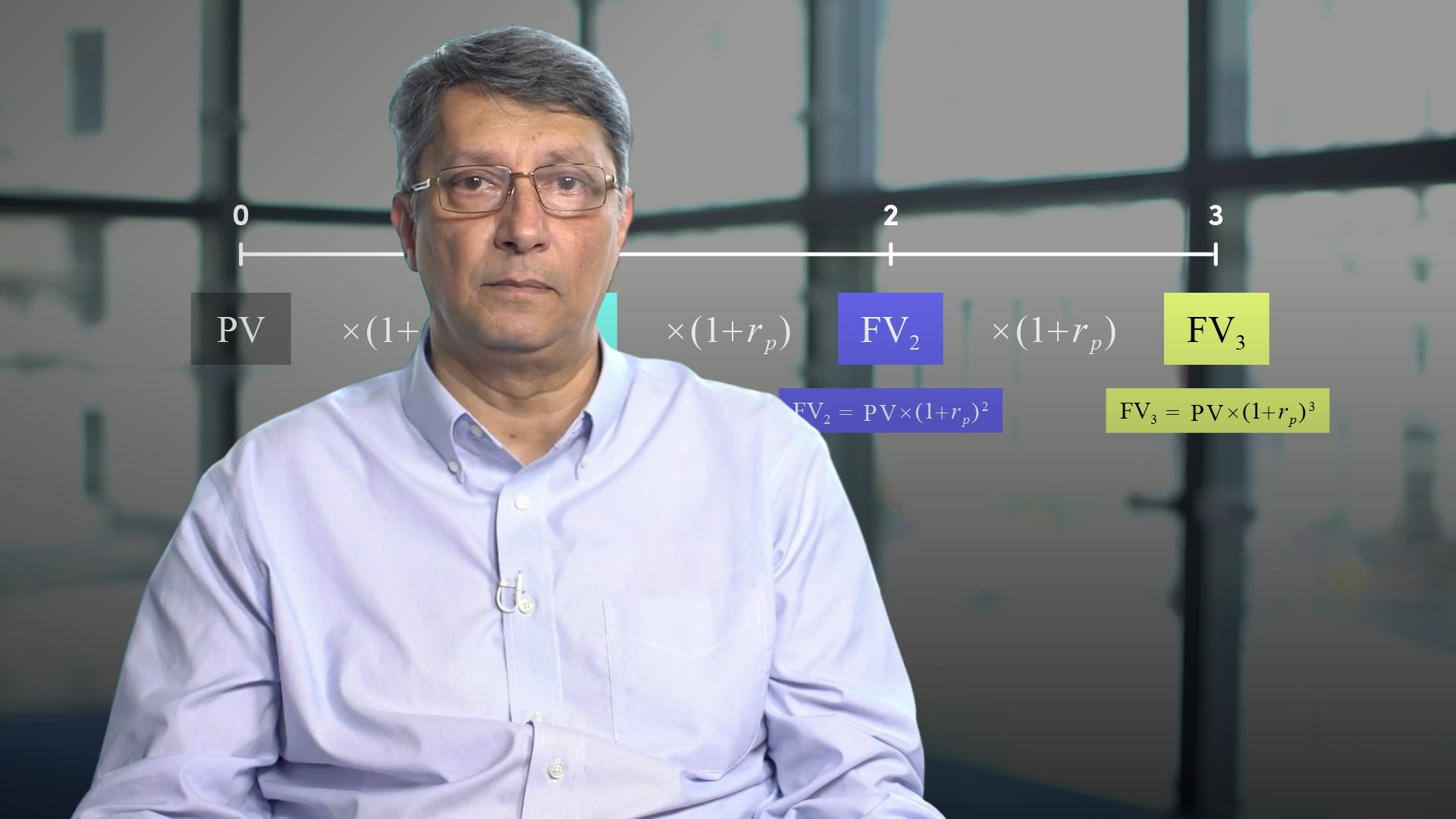06:0805:28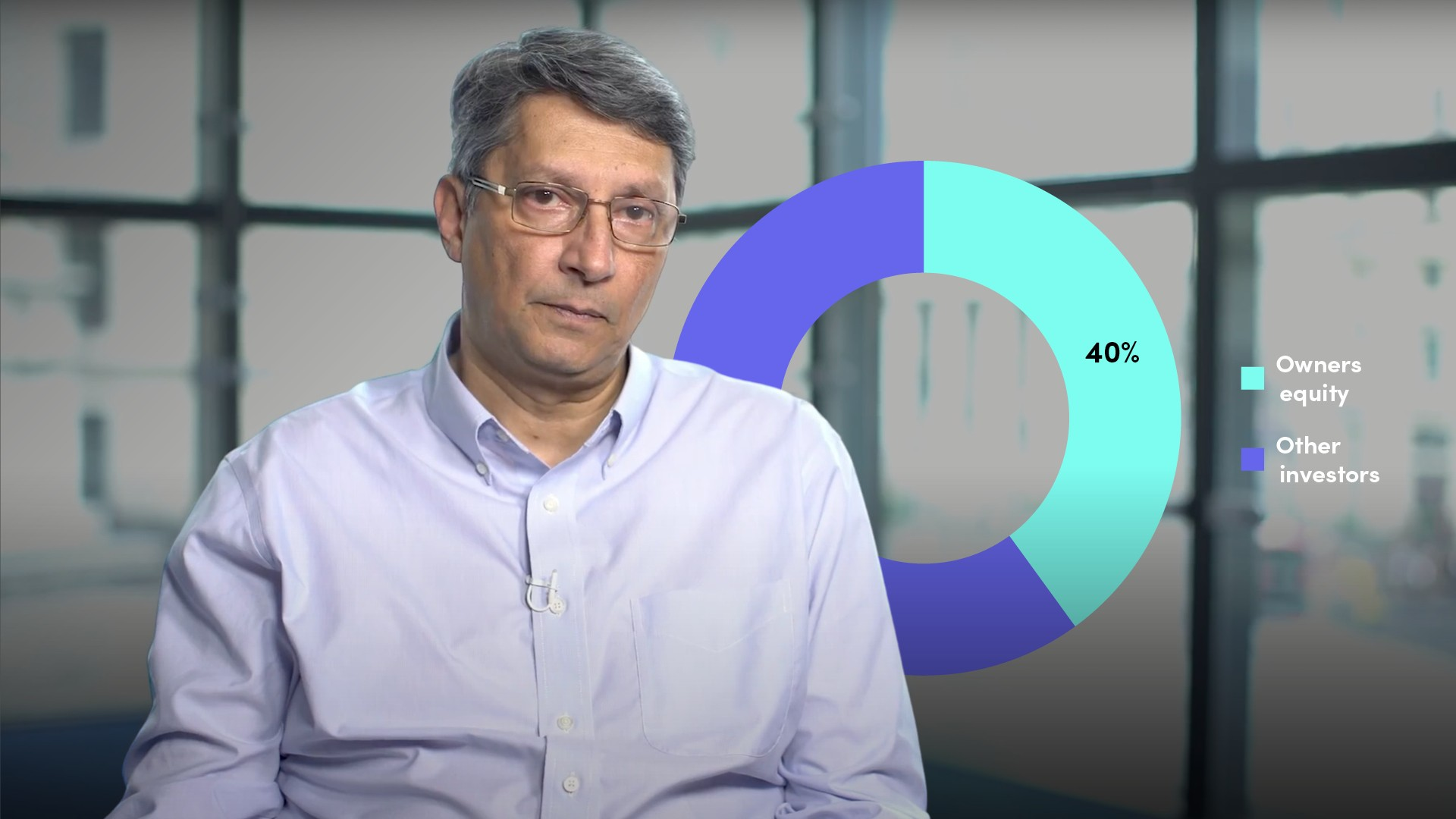05:23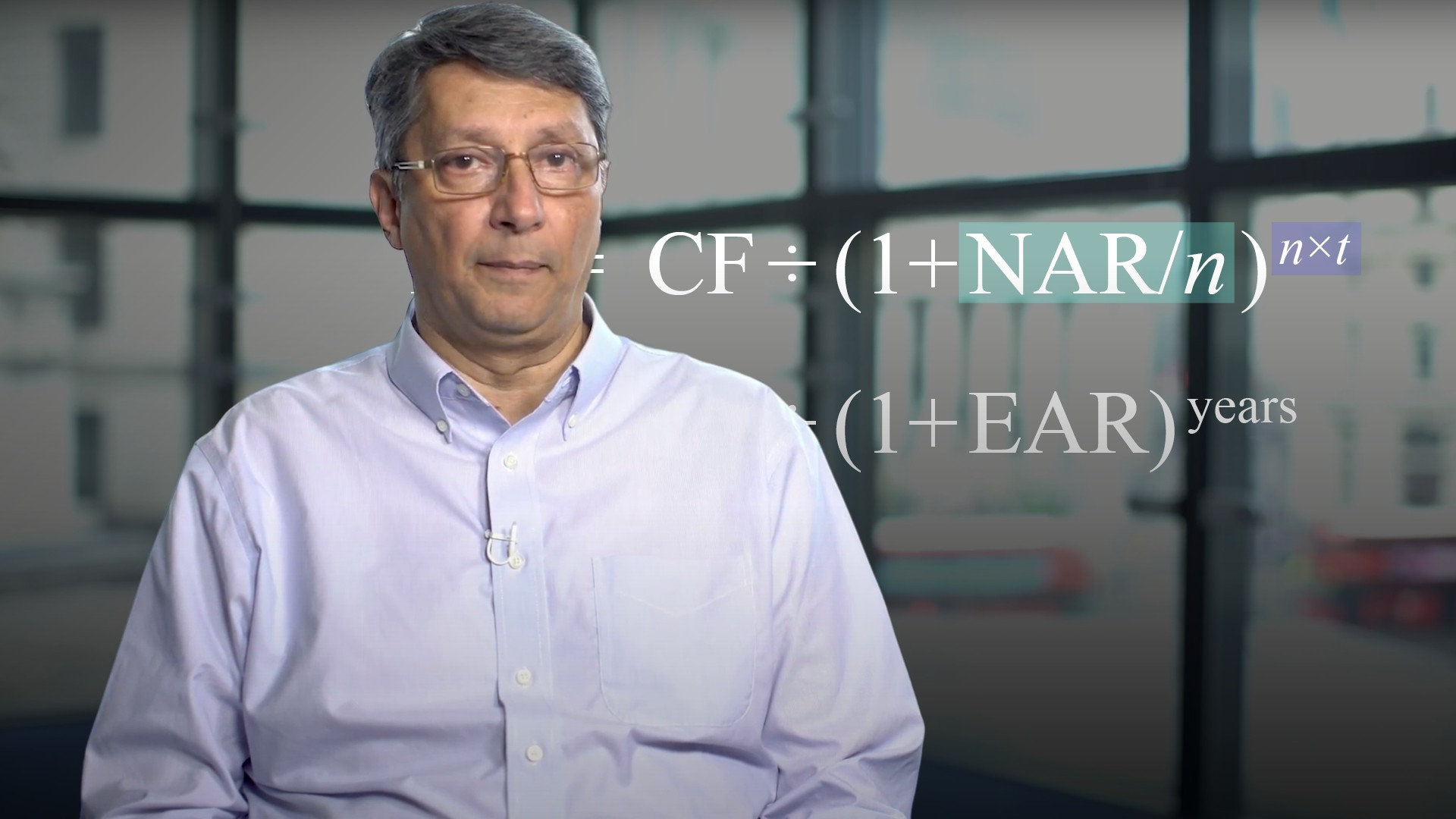04:04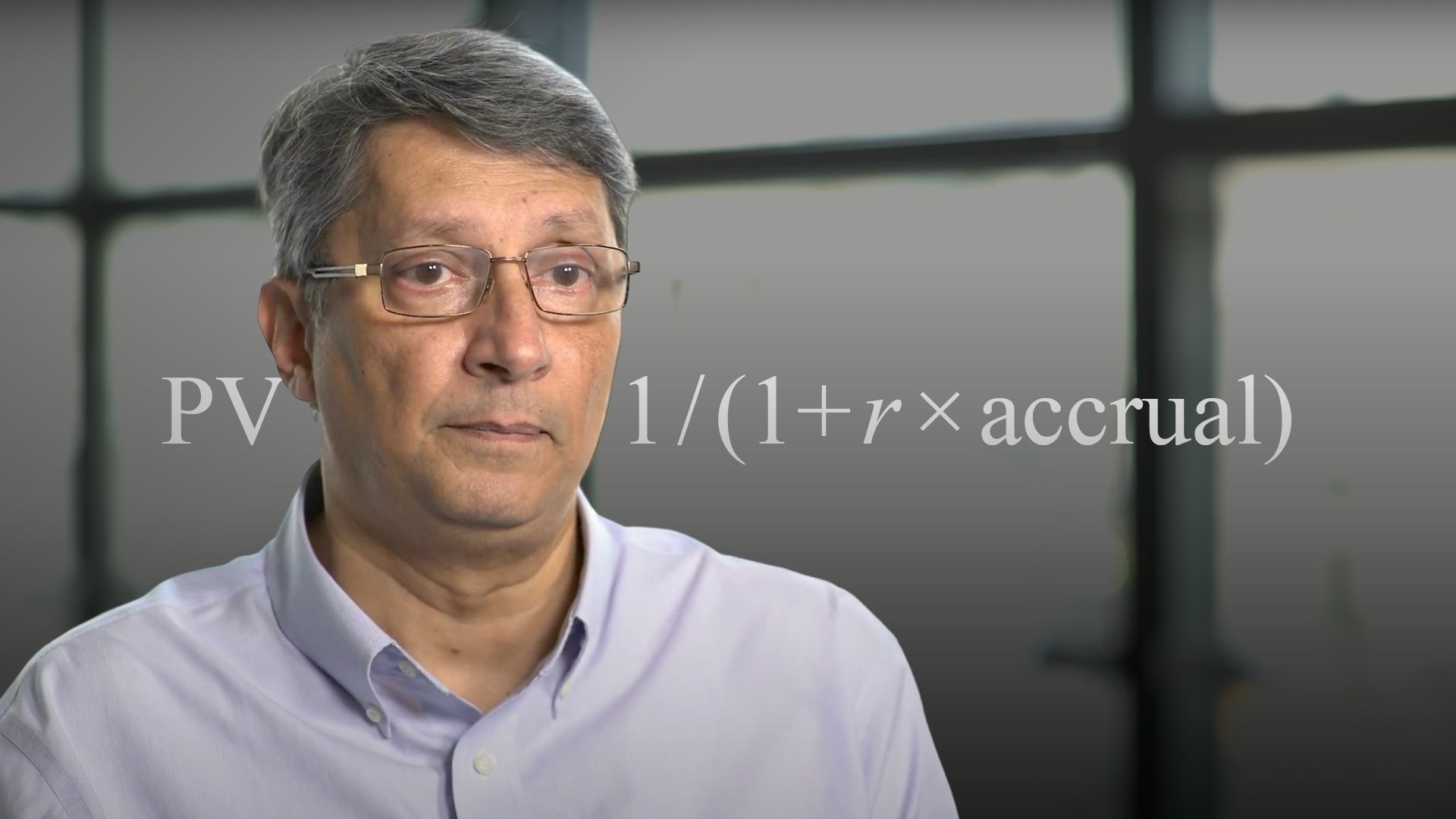03:46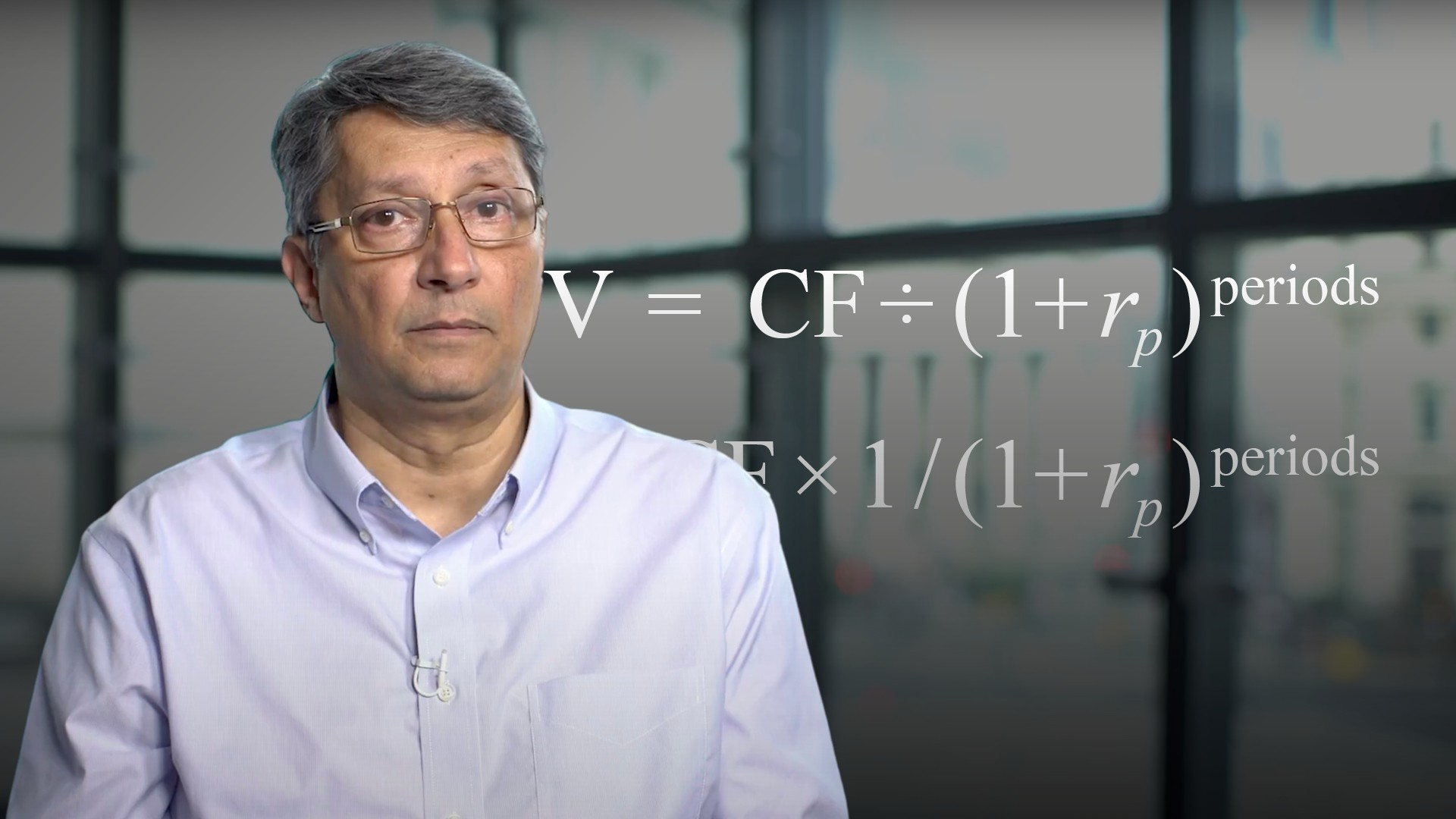04:19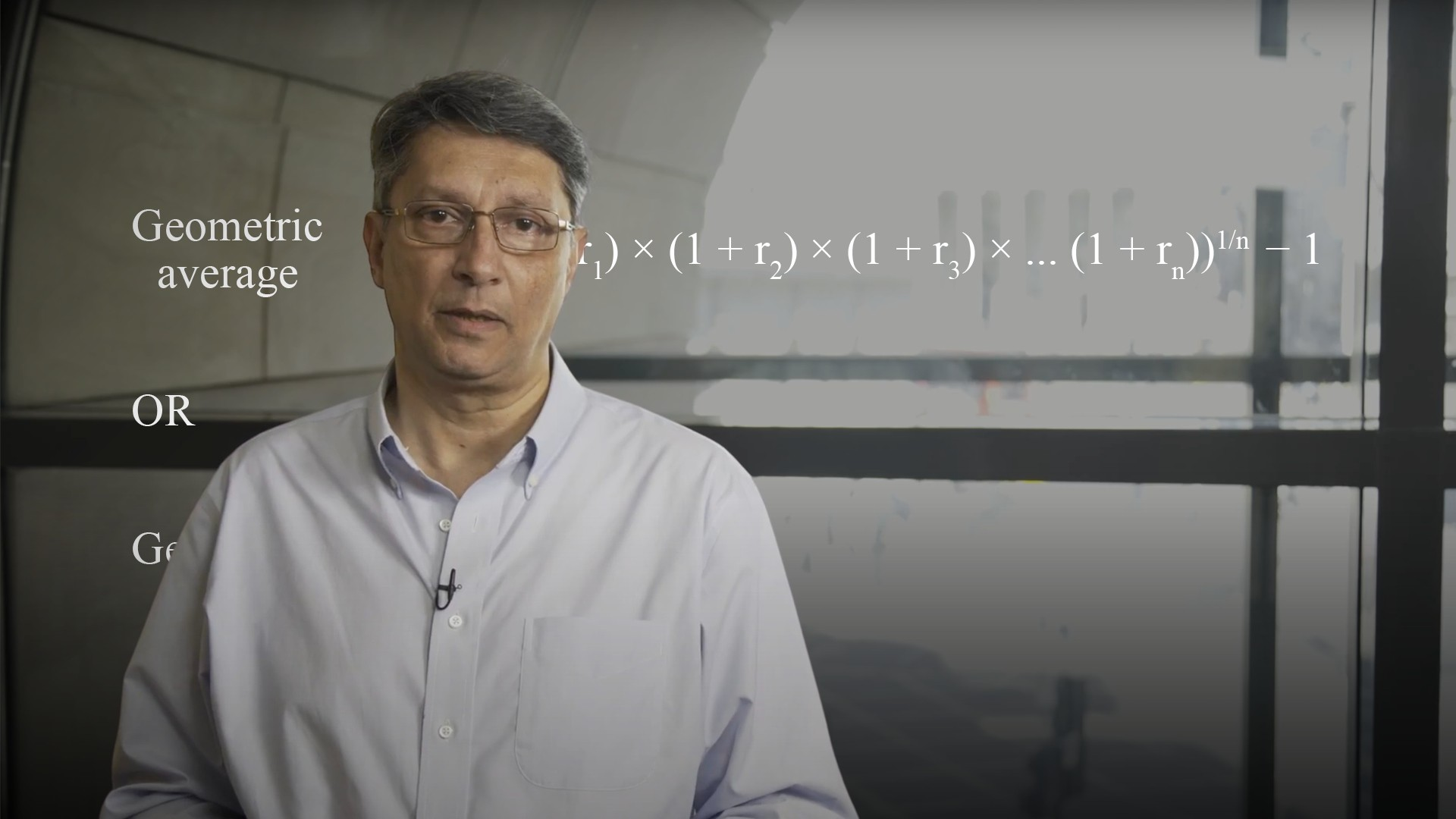04:41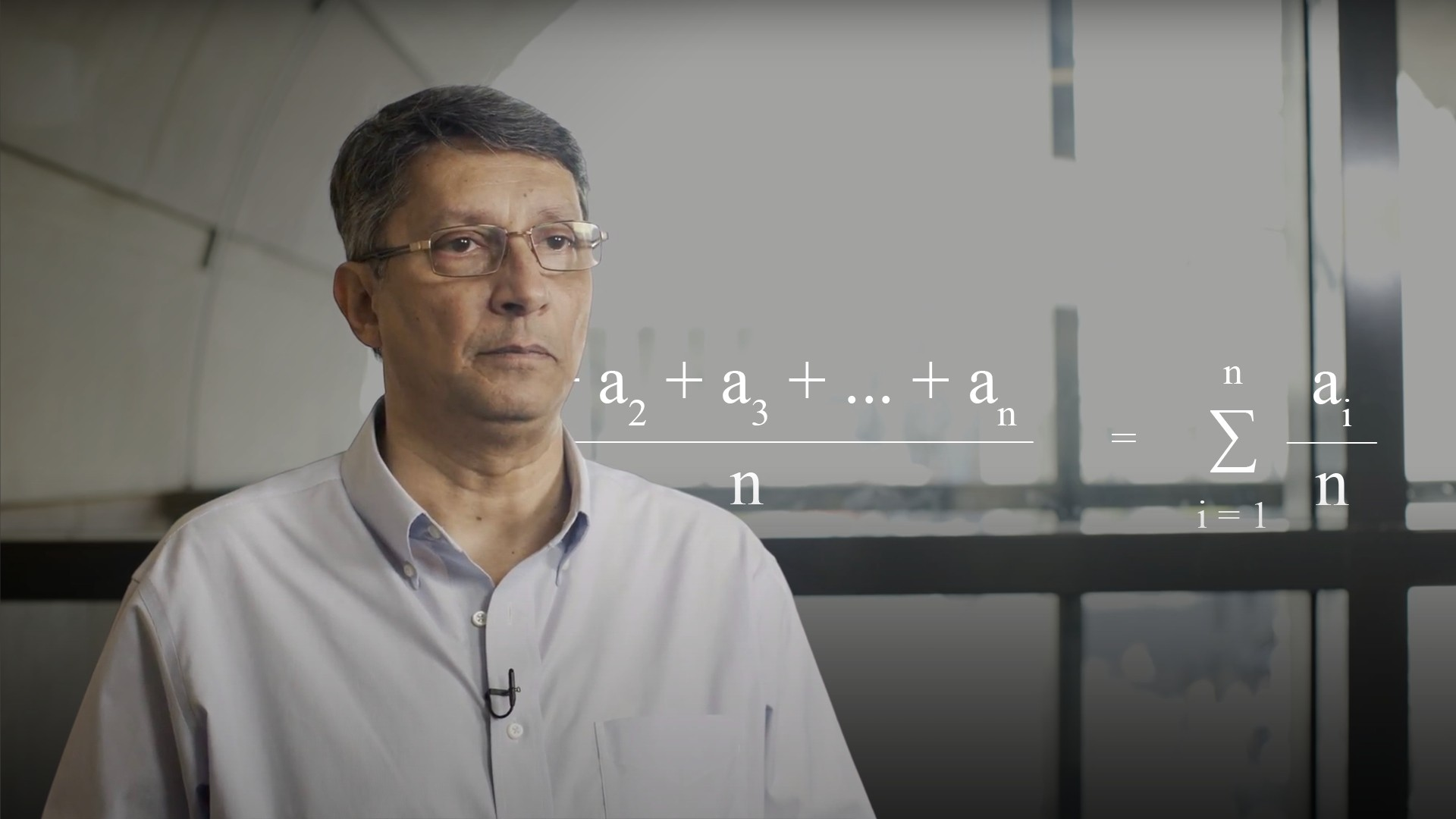04:1605:24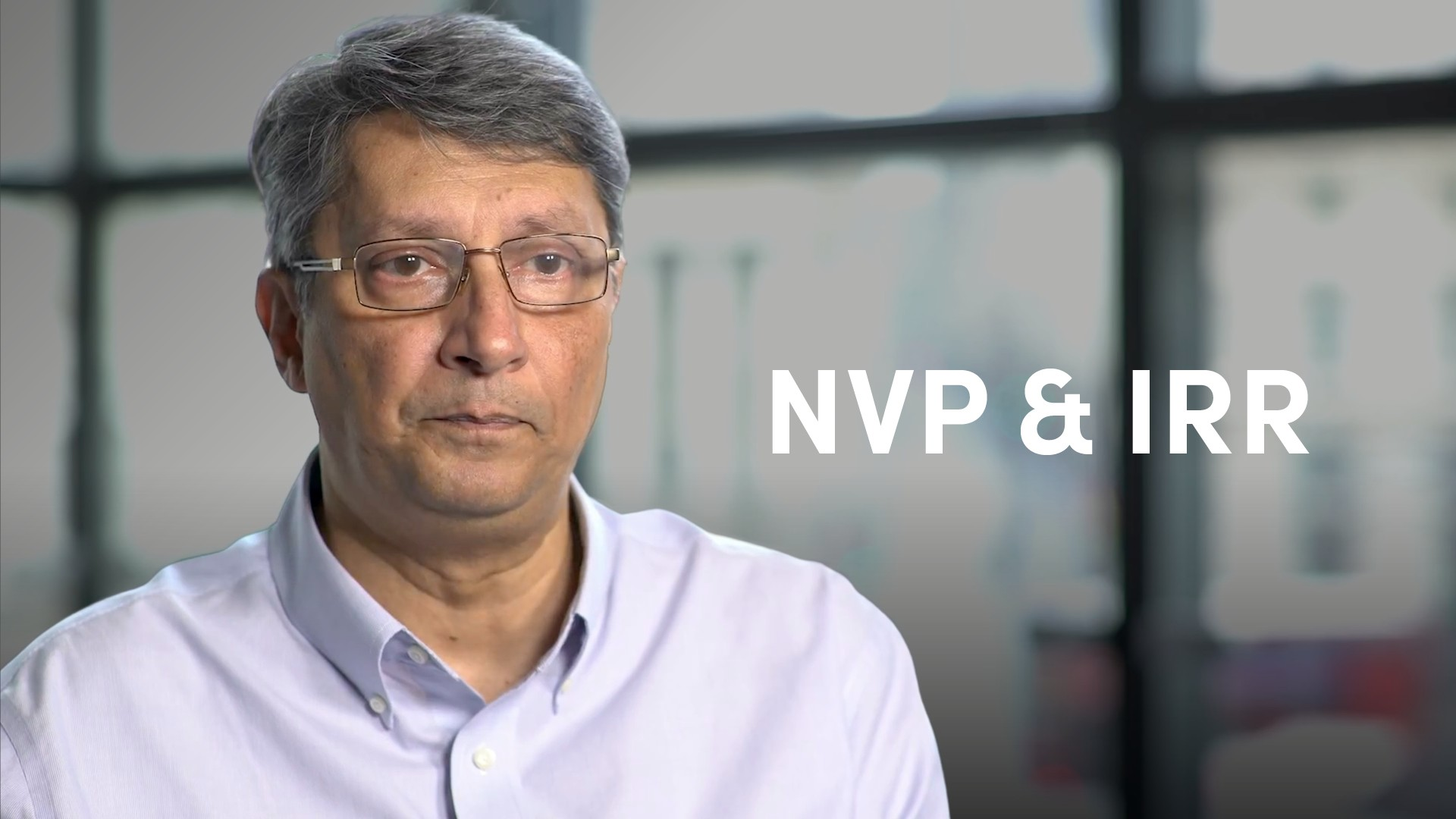05:39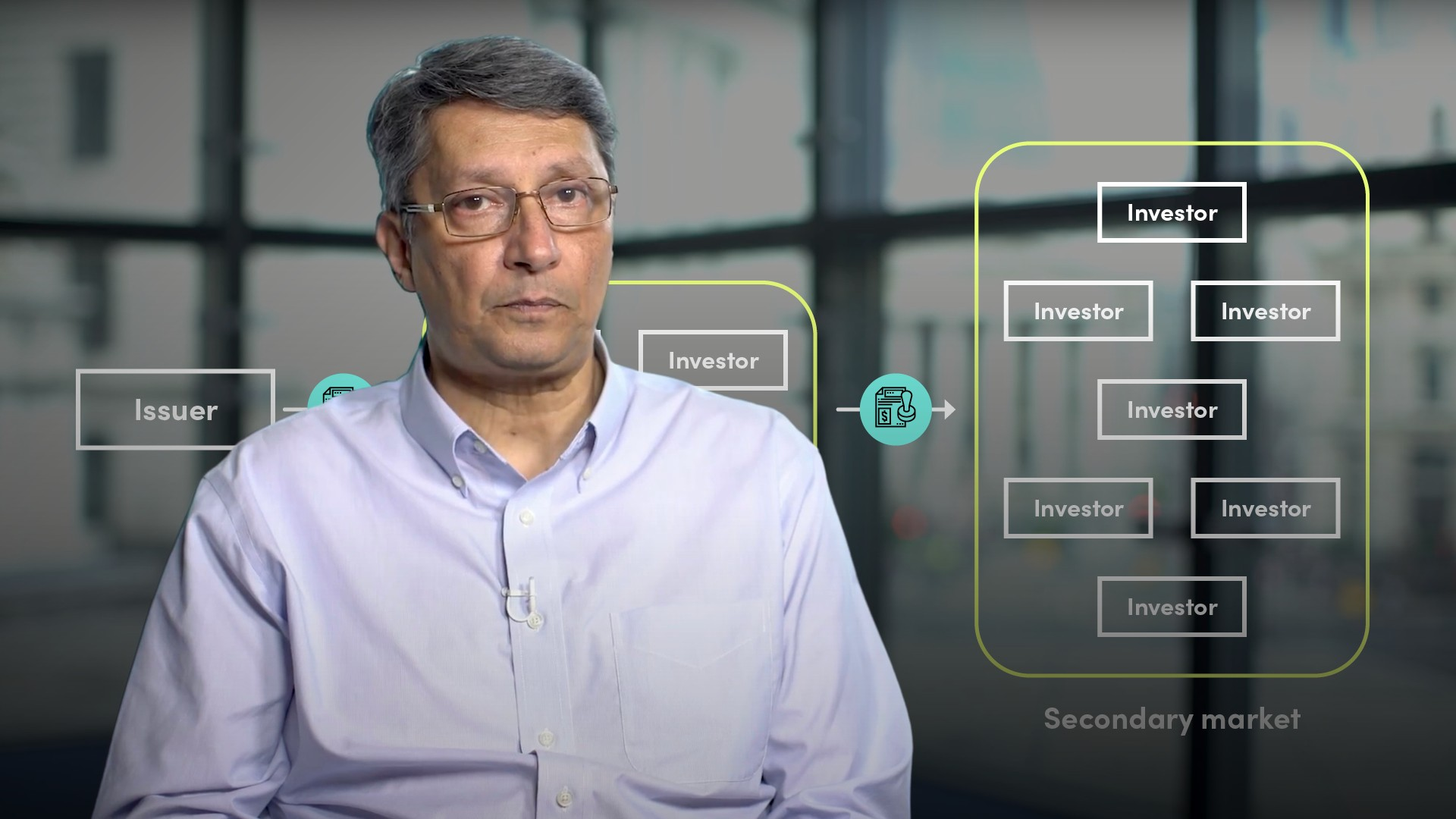06:13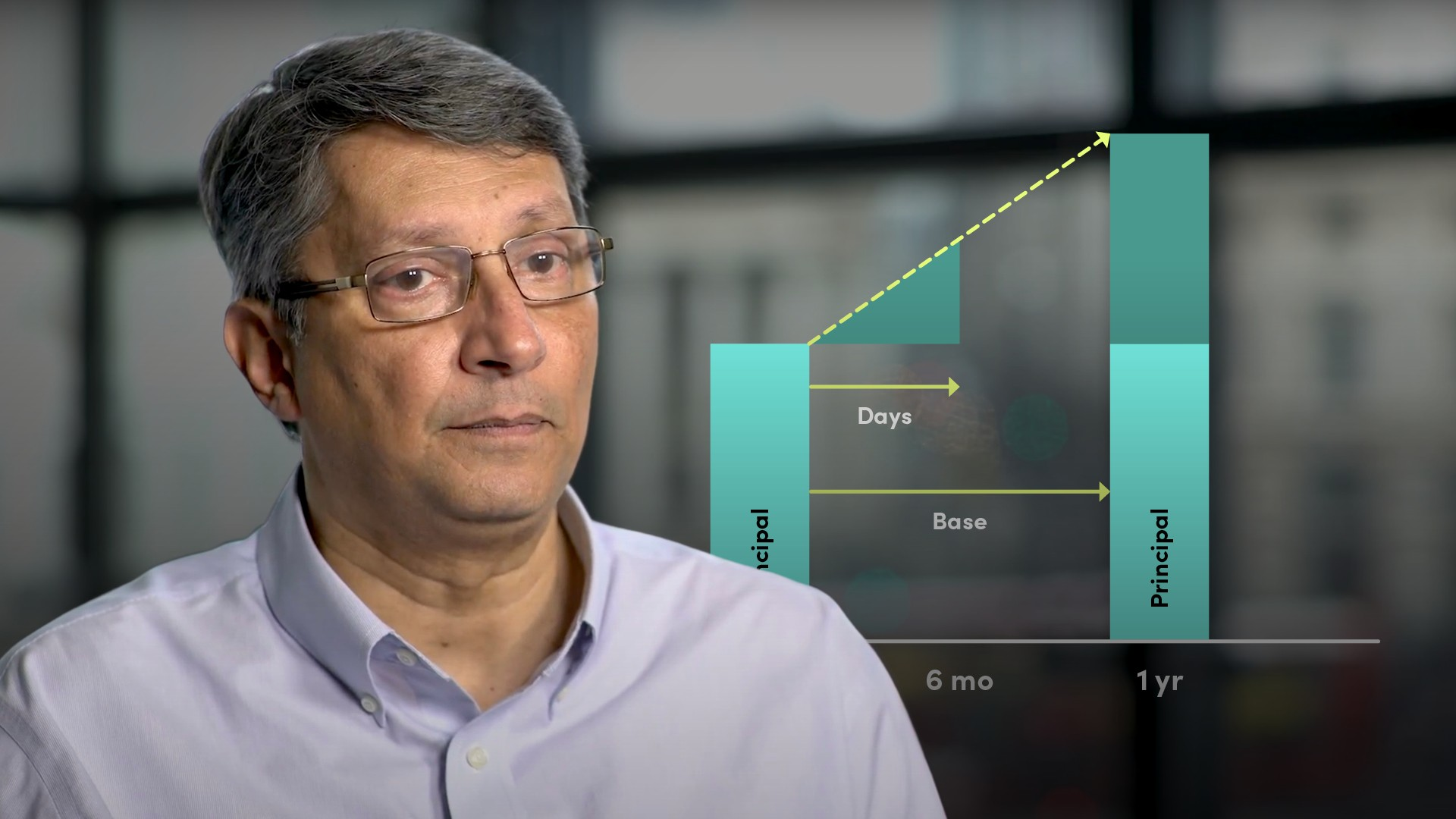05:27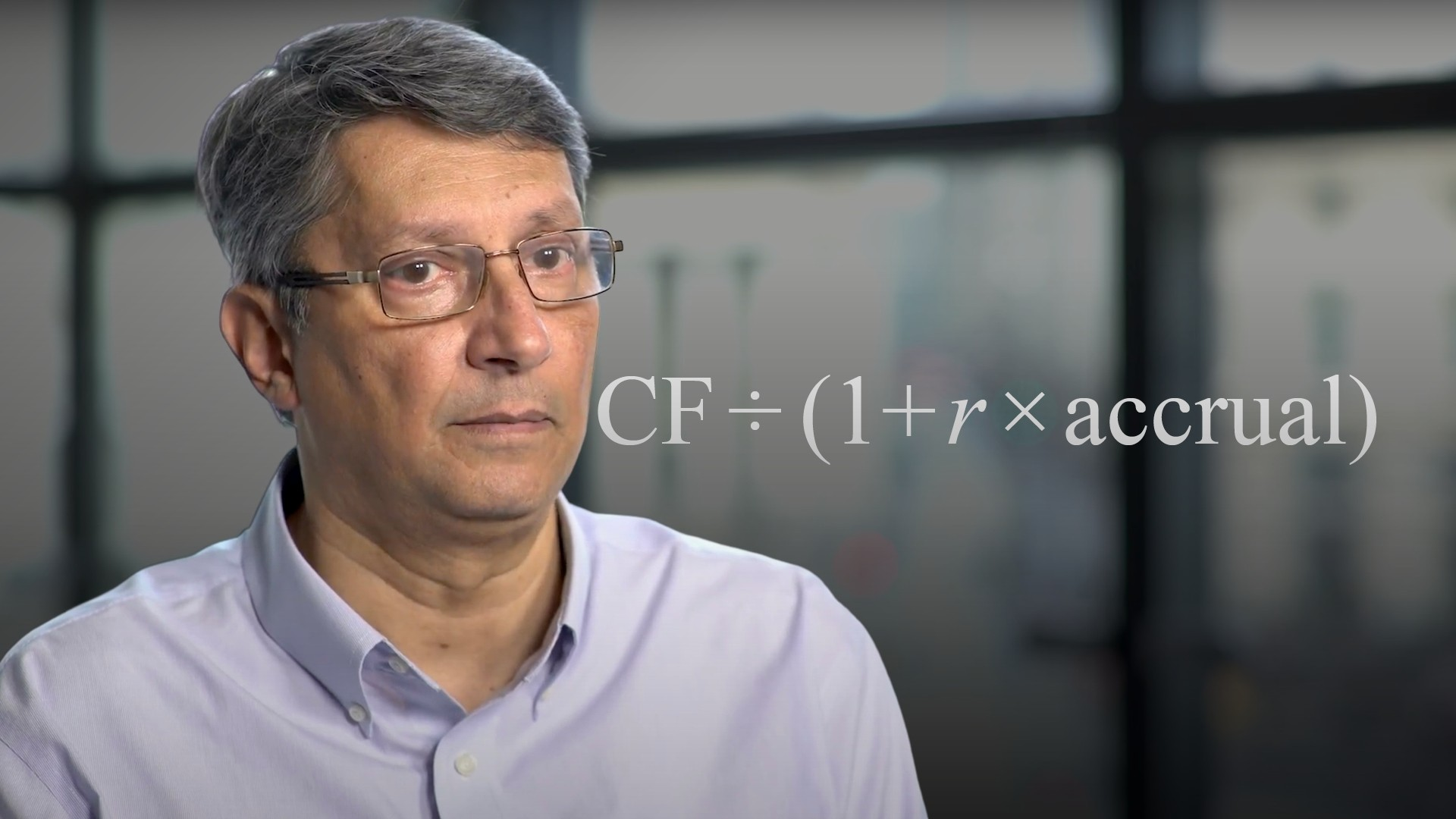03:55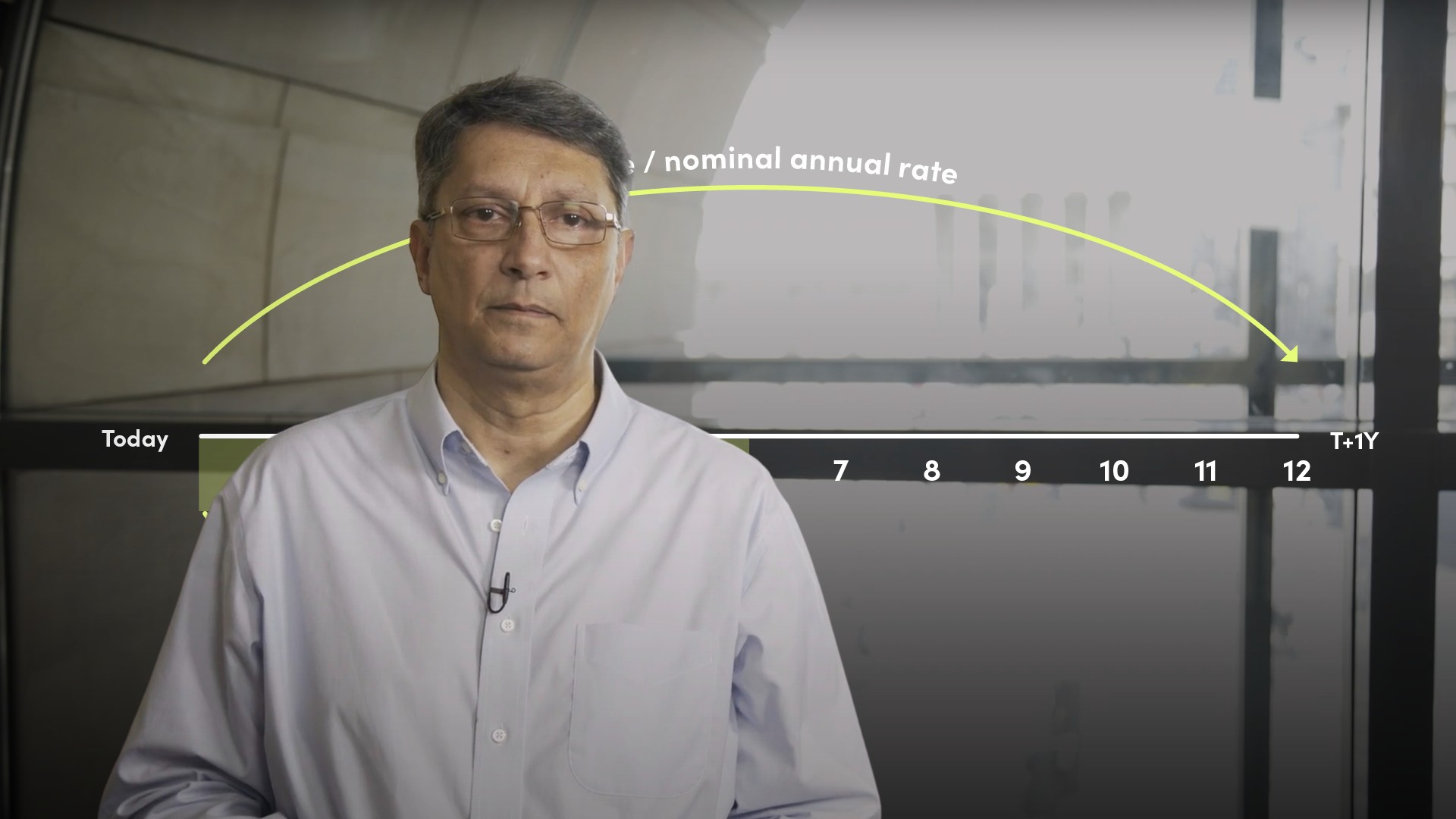07:58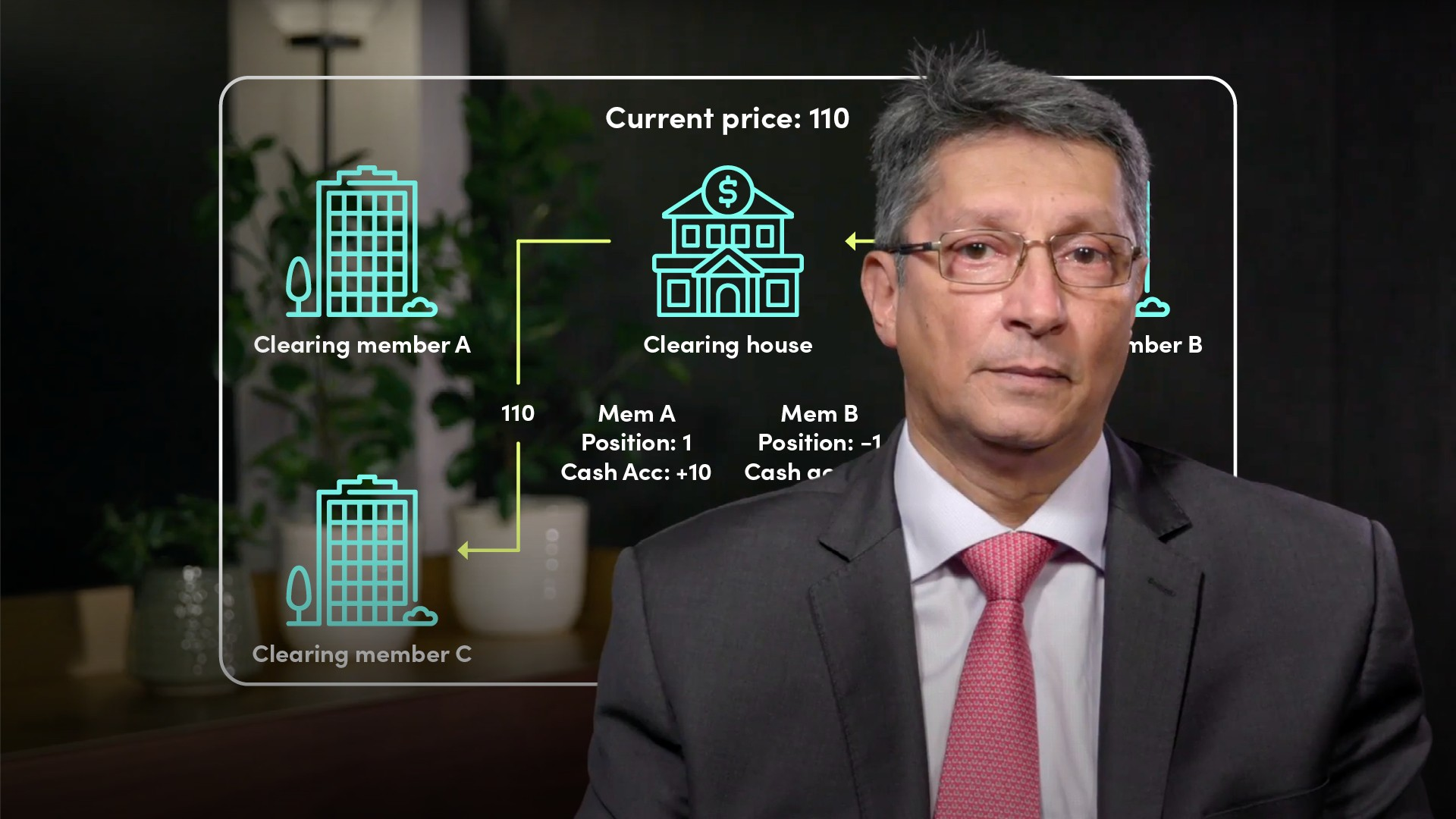05:22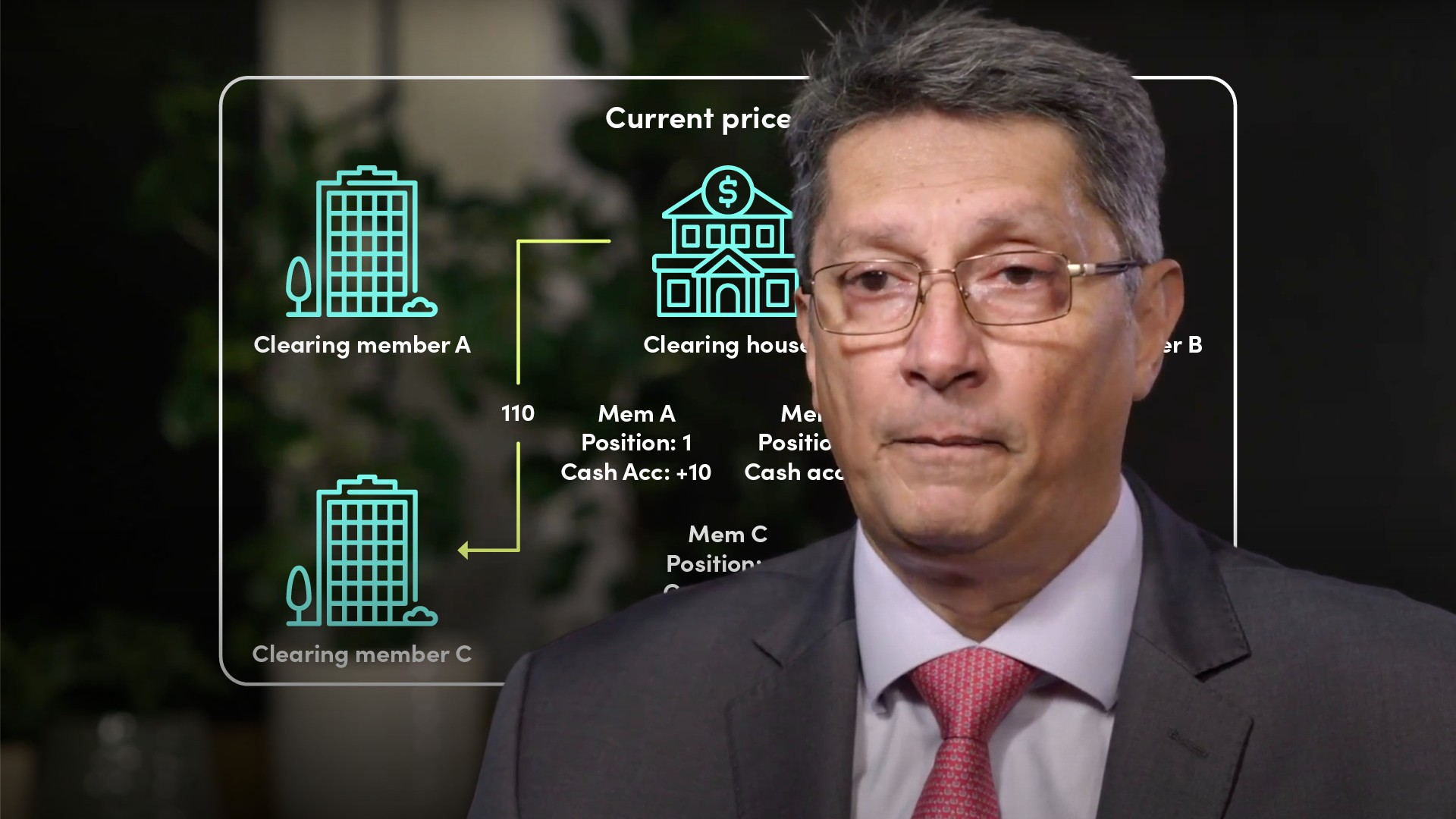05:14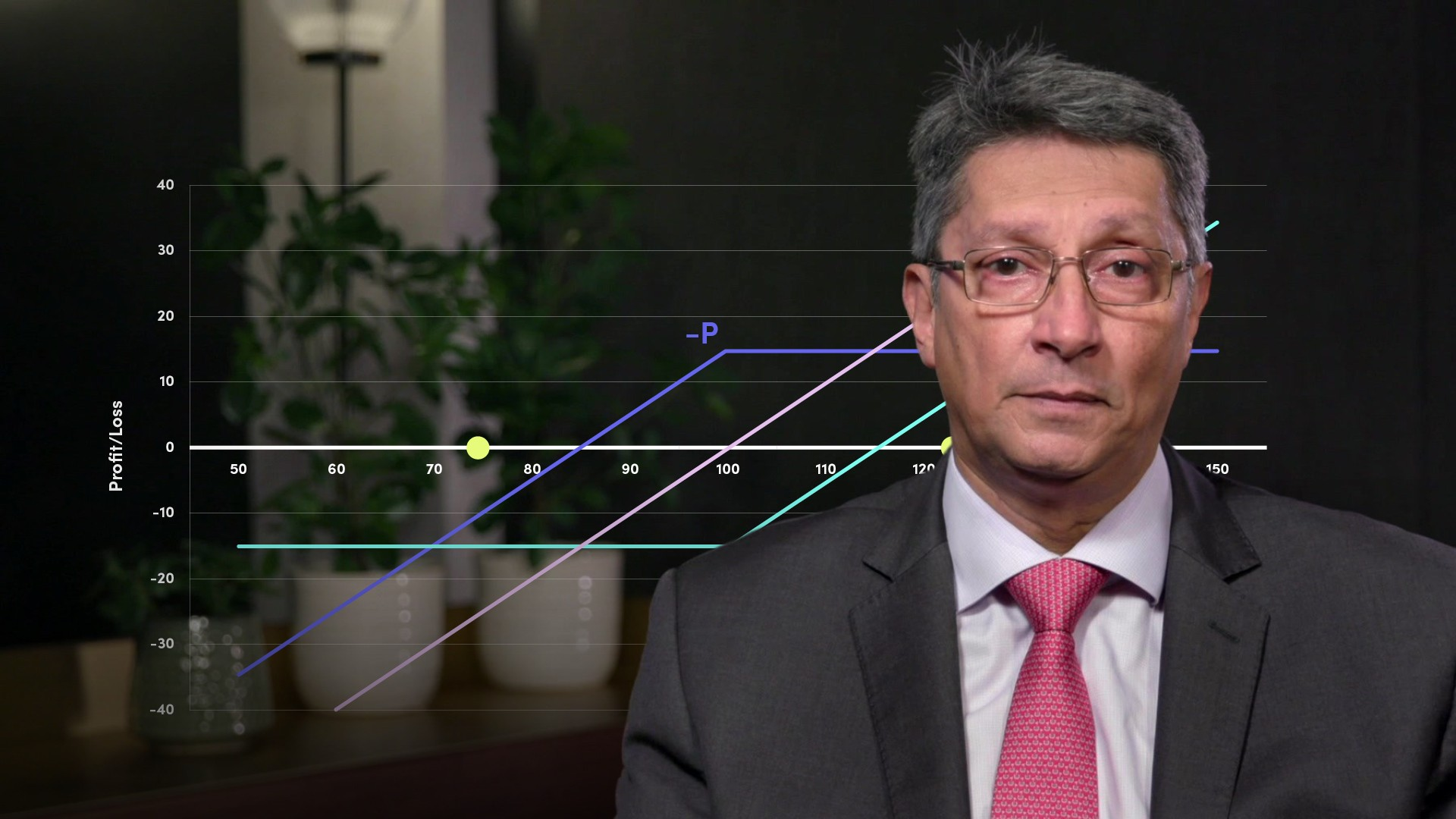06:01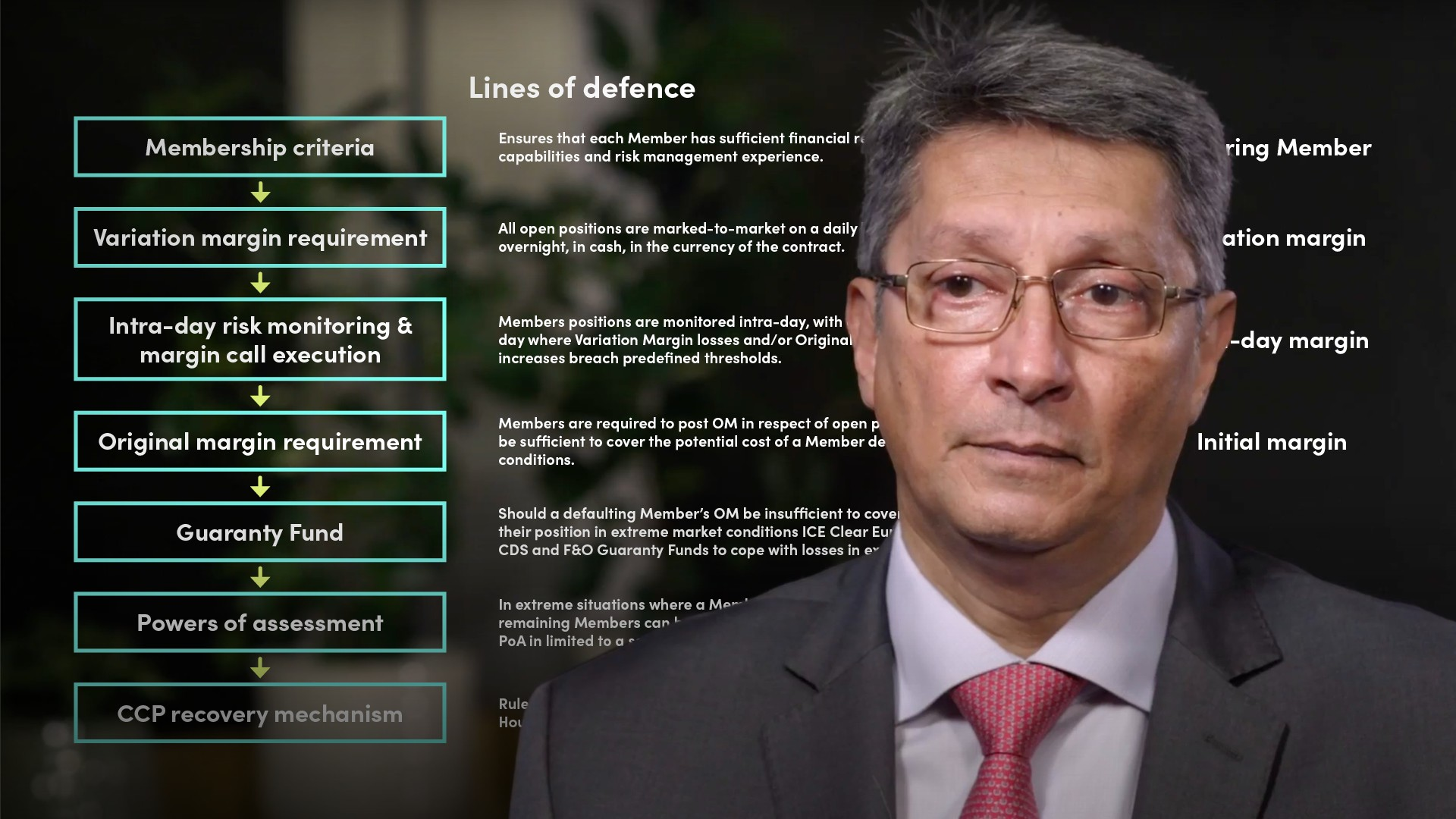06:1306:23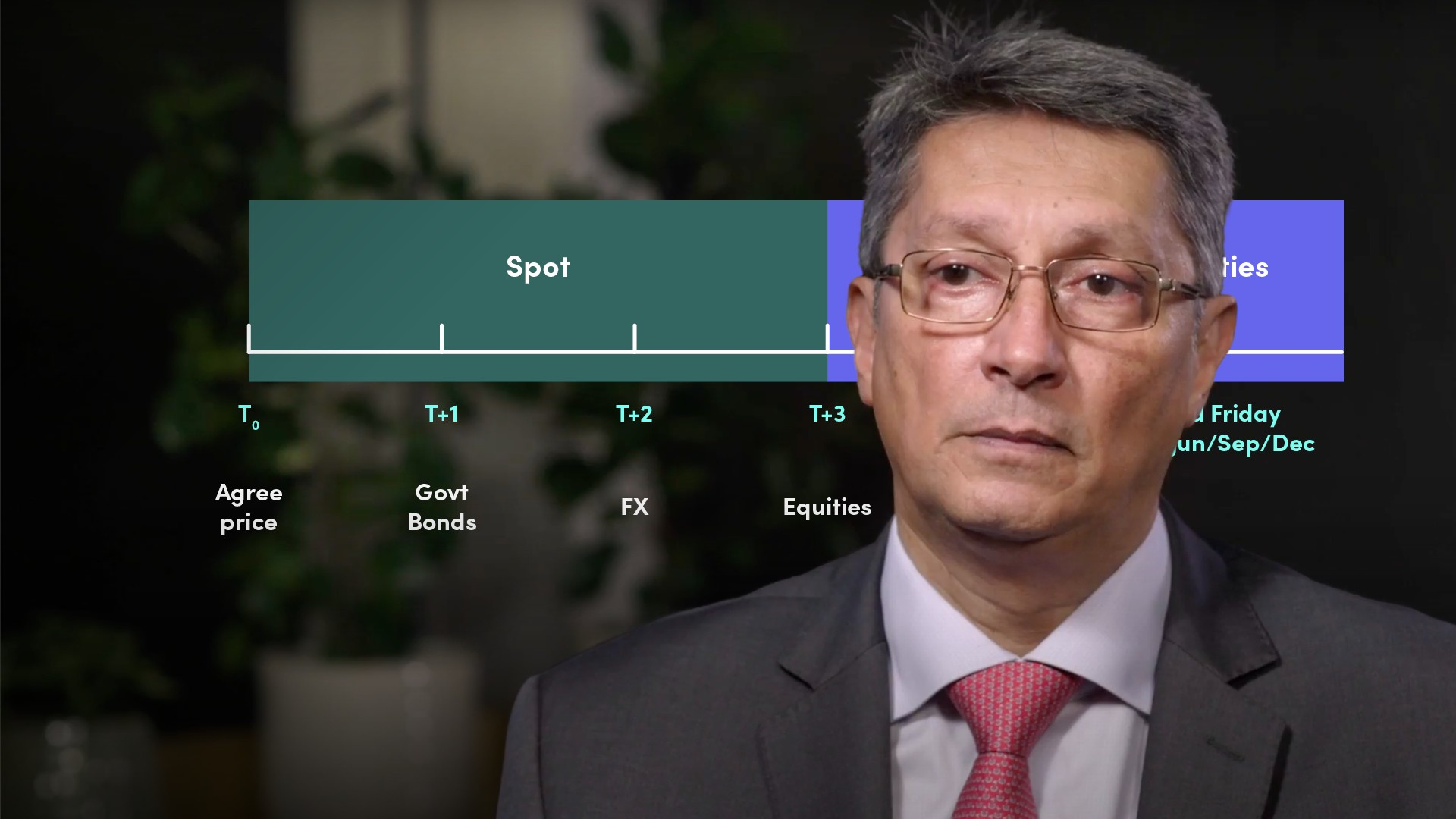05:3807:23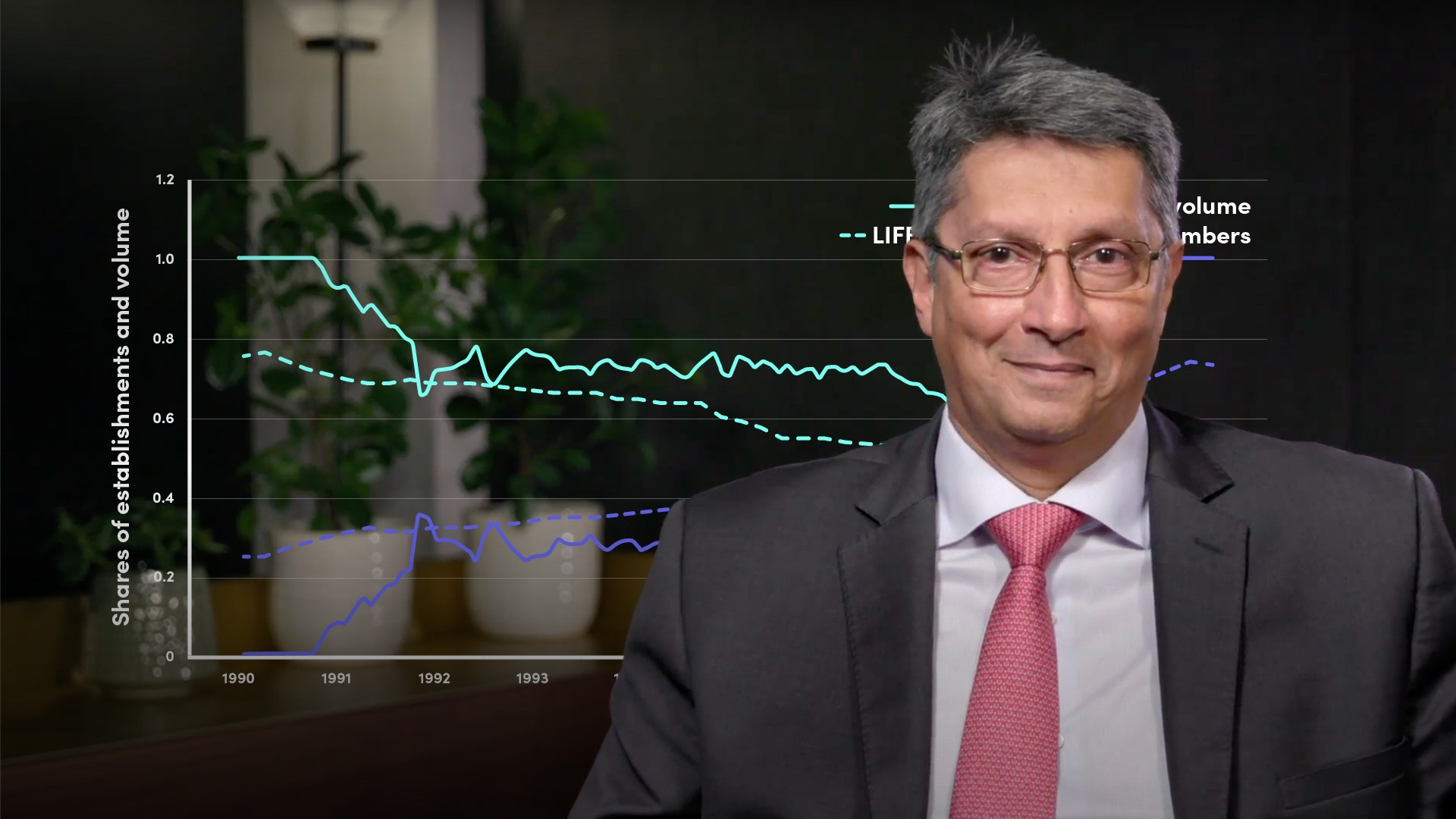04:01

### Accredited by